# RD Sharma Solutions for Class 11 Maths Chapter 6 Graphs of Trigonometric Functions

## RD Sharma Solutions Class 11 Maths Chapter 6 – Free PDF Download

RD Sharma Solutions for Class 11 Maths Chapter 6 – Graphs of Trigonometric Functions is provided here. In the previous chapters, we have learnt that all trigonometric functions are periodic functions, so we shall draw their graphs on the intervals of lengths equal to their periods. In order to enhance the performance of students in Class 11 exam, we at BYJU’S have formulated the RD Sharma Class 11 Solutions for Maths under. Exercise-wise problems are solved in a step by step method to help students understand the concepts easily. Students are advised to practice the solutions on a regular basis to obtain good marks in their exams. The RD Sharma Solutions are available in the PDF format, which can be downloaded easily from the links provided below.

RD Sharma Solutions for Class 11 Maths Chapter 6 – Graphs of Trigonometric Functions contains three exercises and the RD Sharma Solutions present in this page provide solutions to the questions present in each exercise. Now, let us have a look at the concepts discussed in this chapter.

• Graph of the sine function.
• Graph of the cosine function.
• Graph of the tangent function.
• Graph of cosecant function.
• Graph of cotangent function.
• Graph of secant function.

## Download the Pdf of RD Sharma Solutions for Class 11 Maths Chapter 6 – Graphs of Trigonometric Functions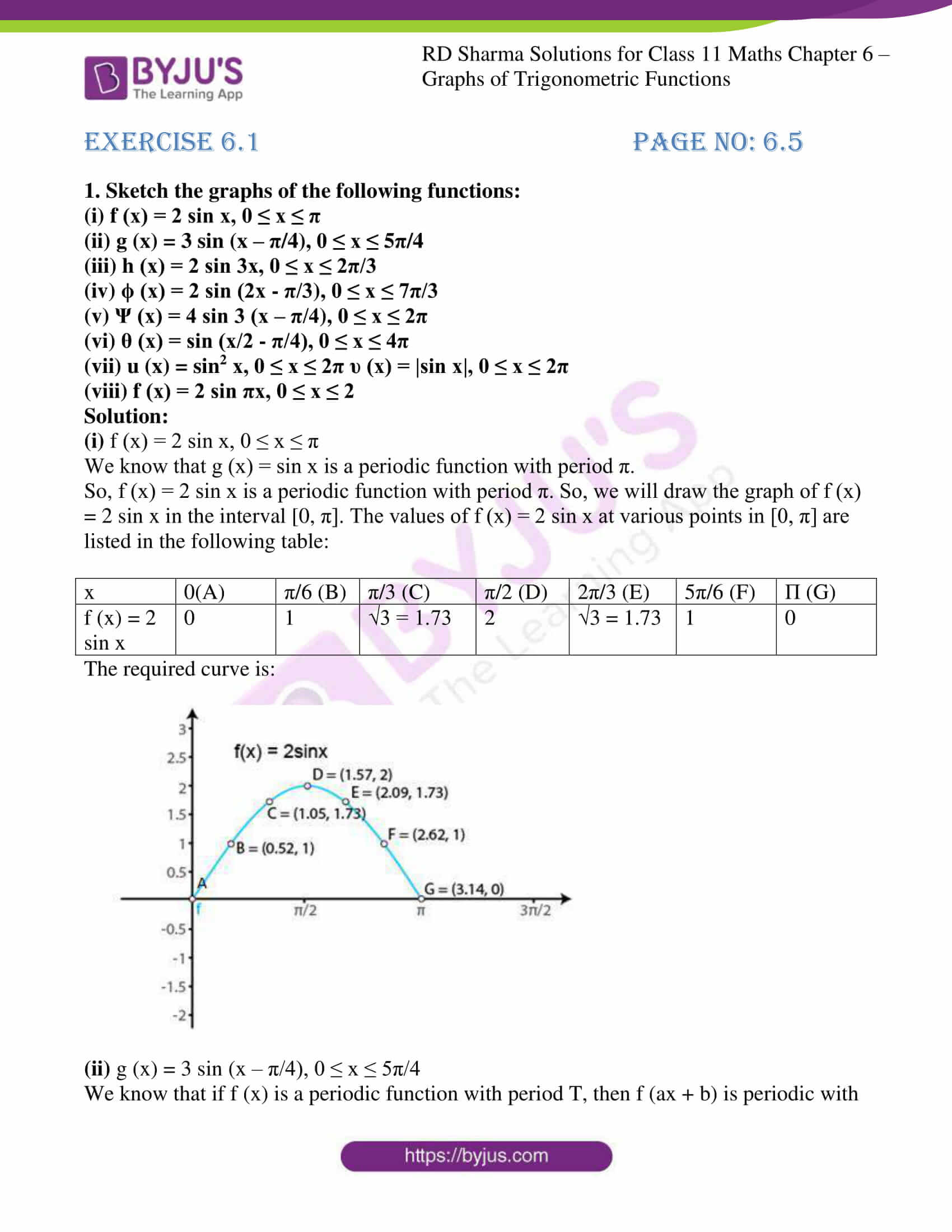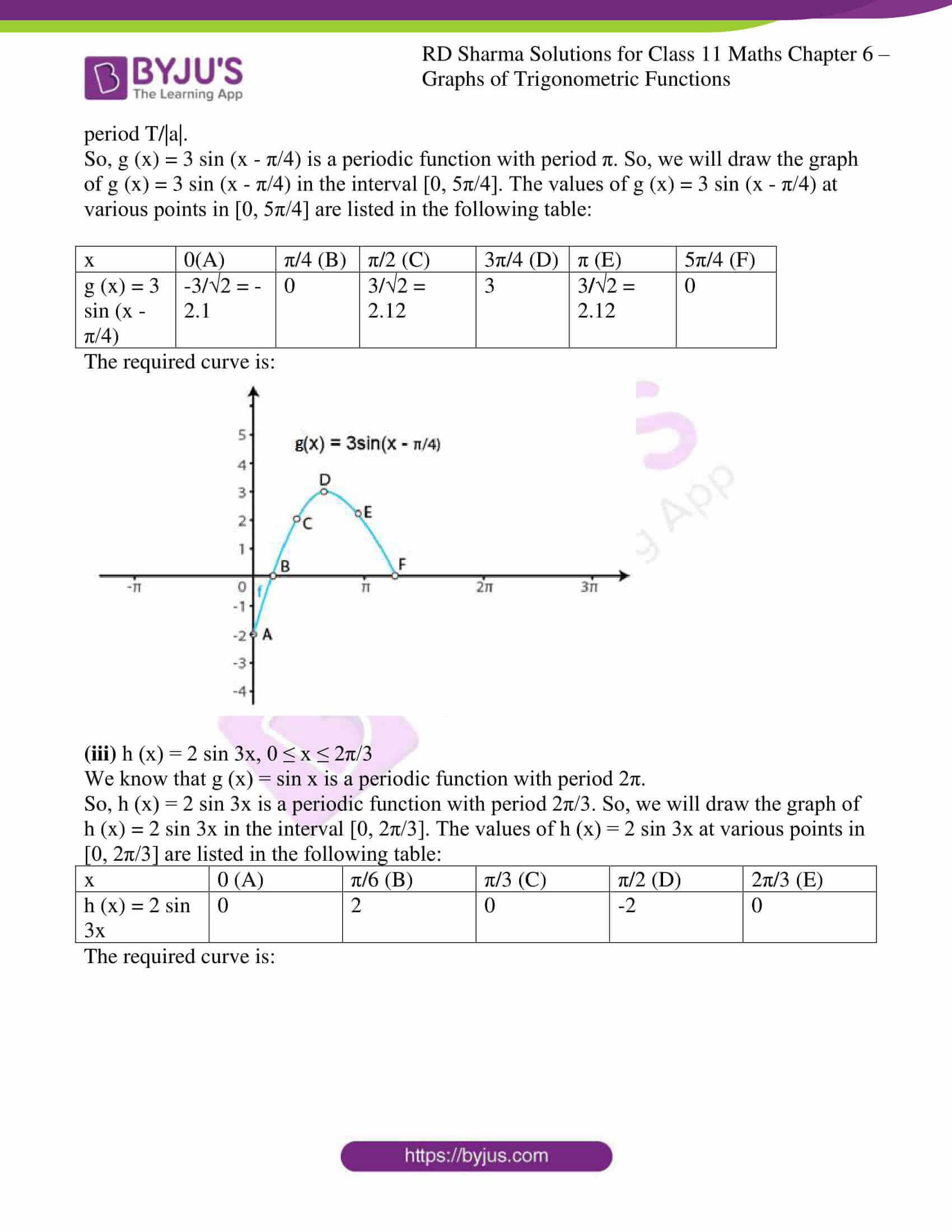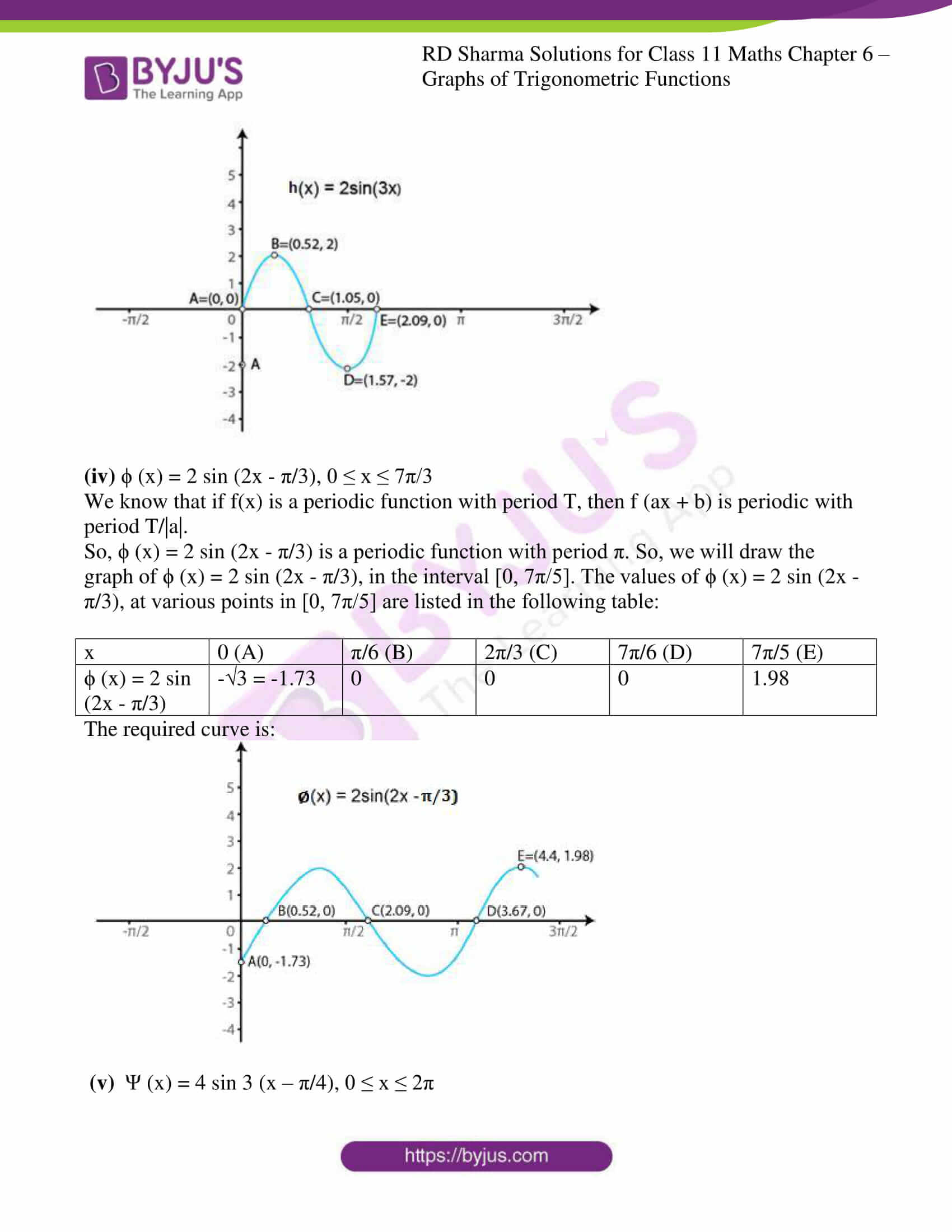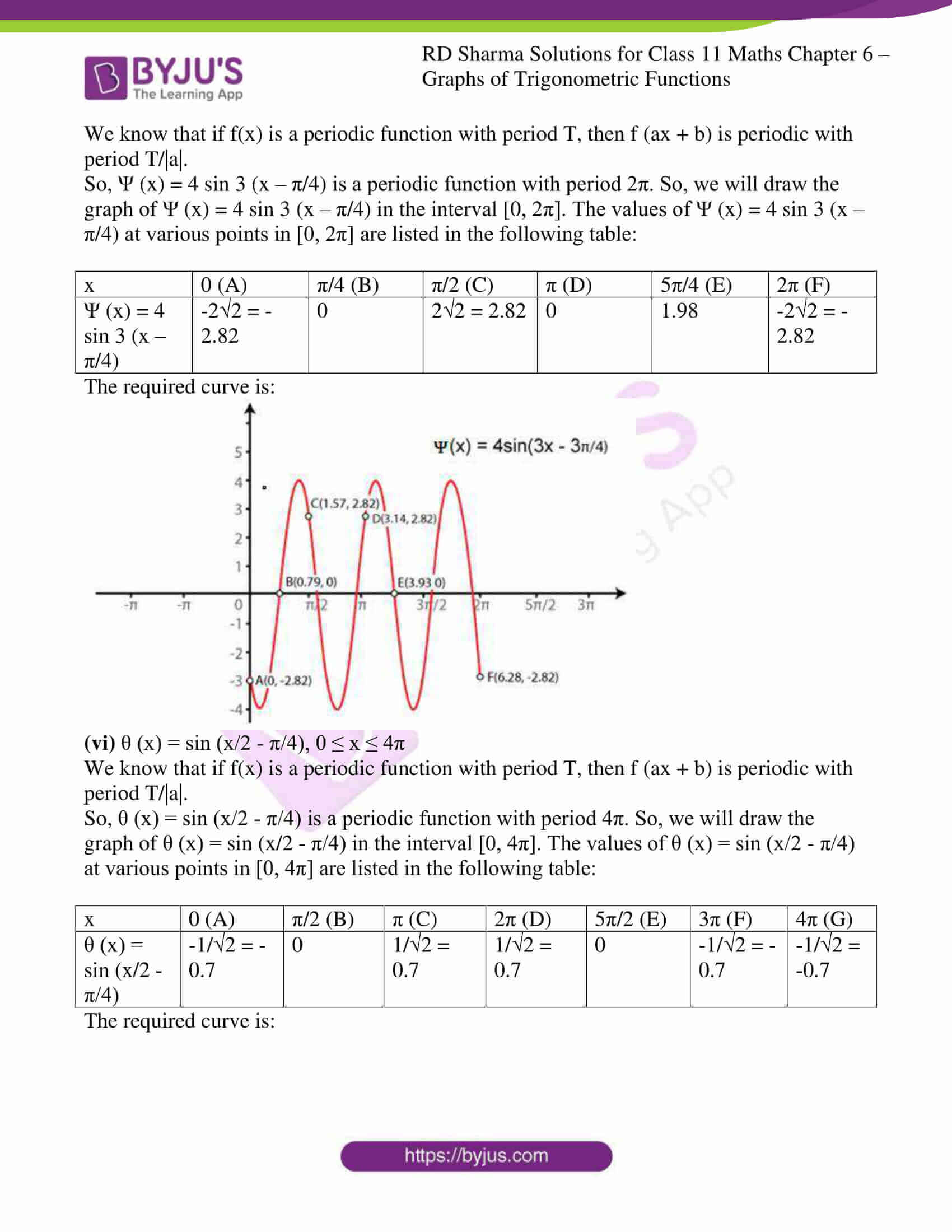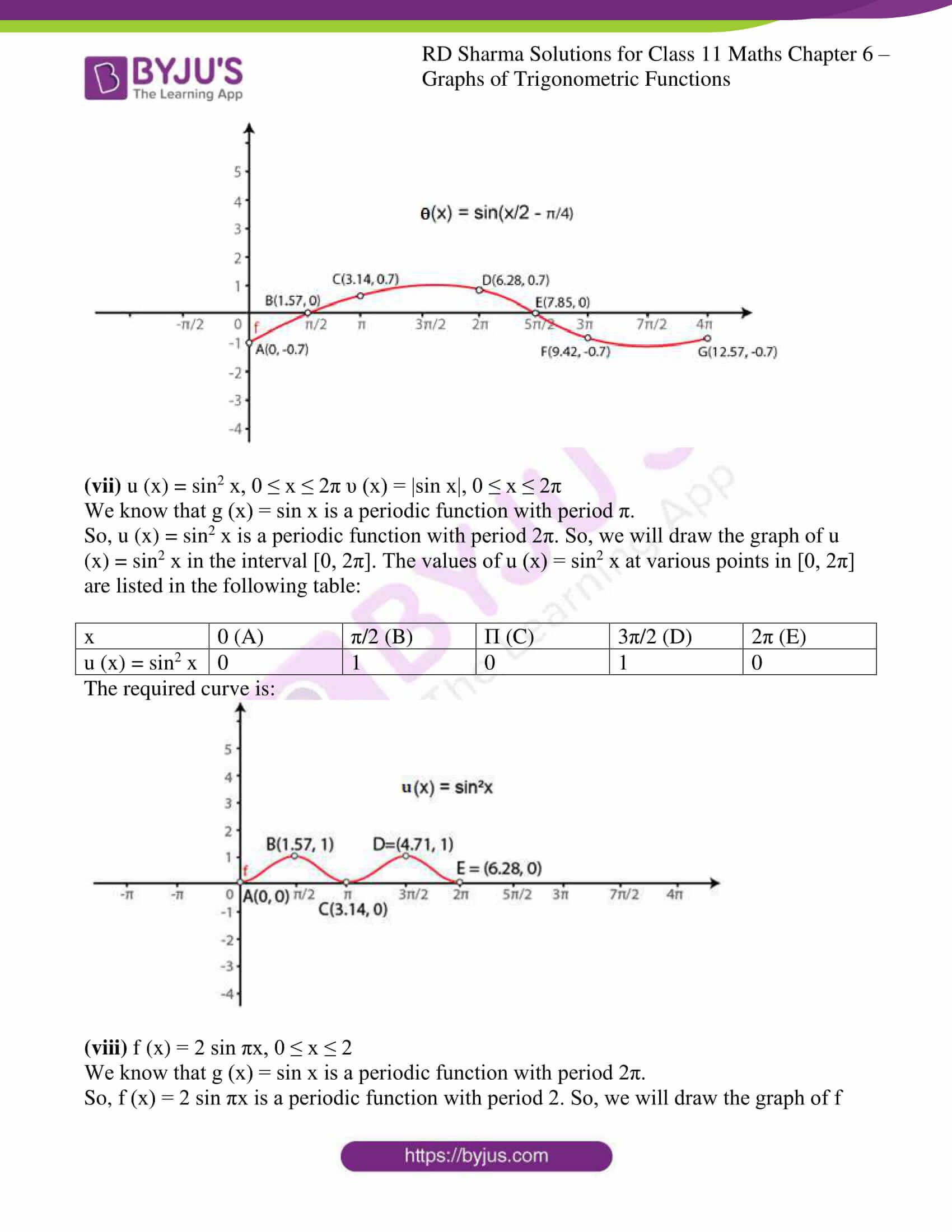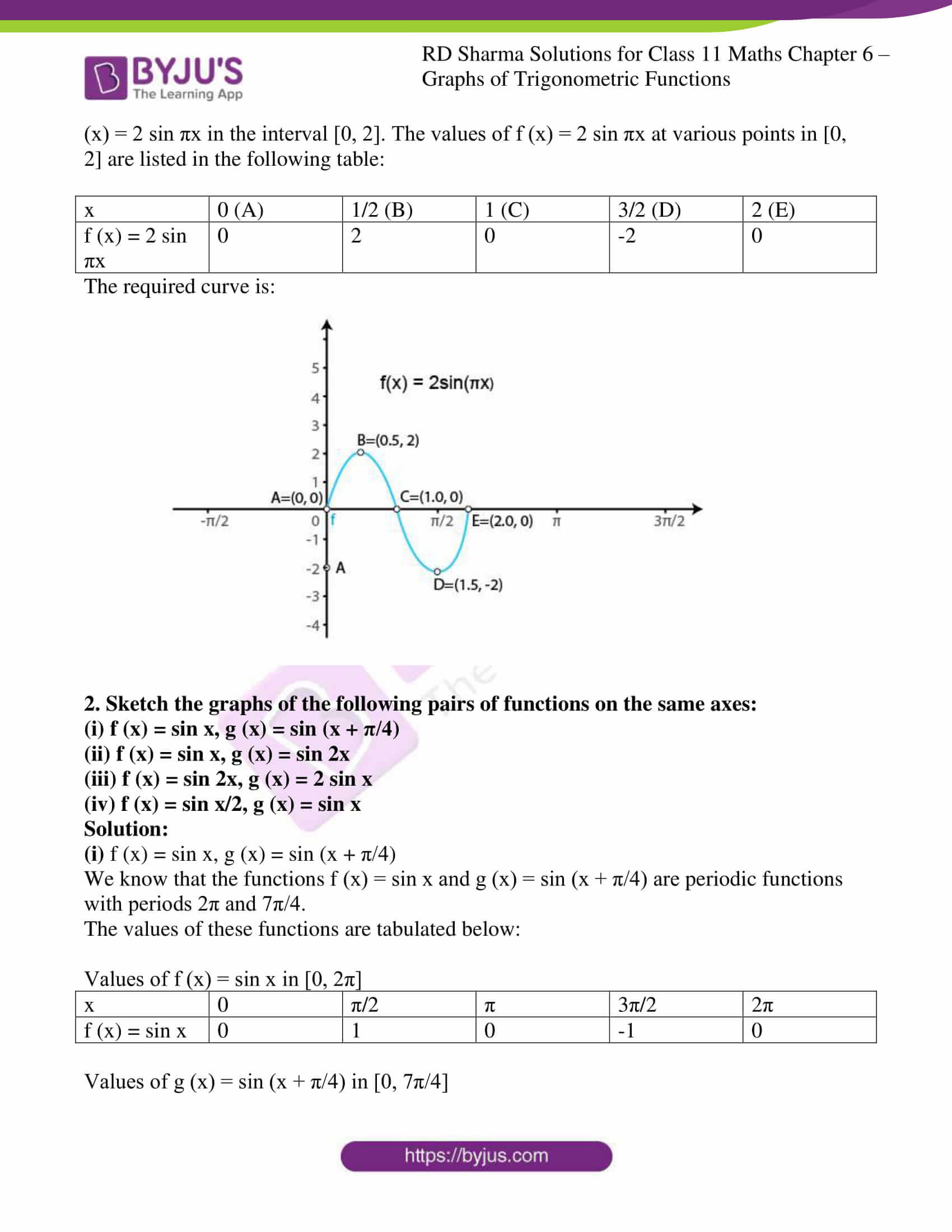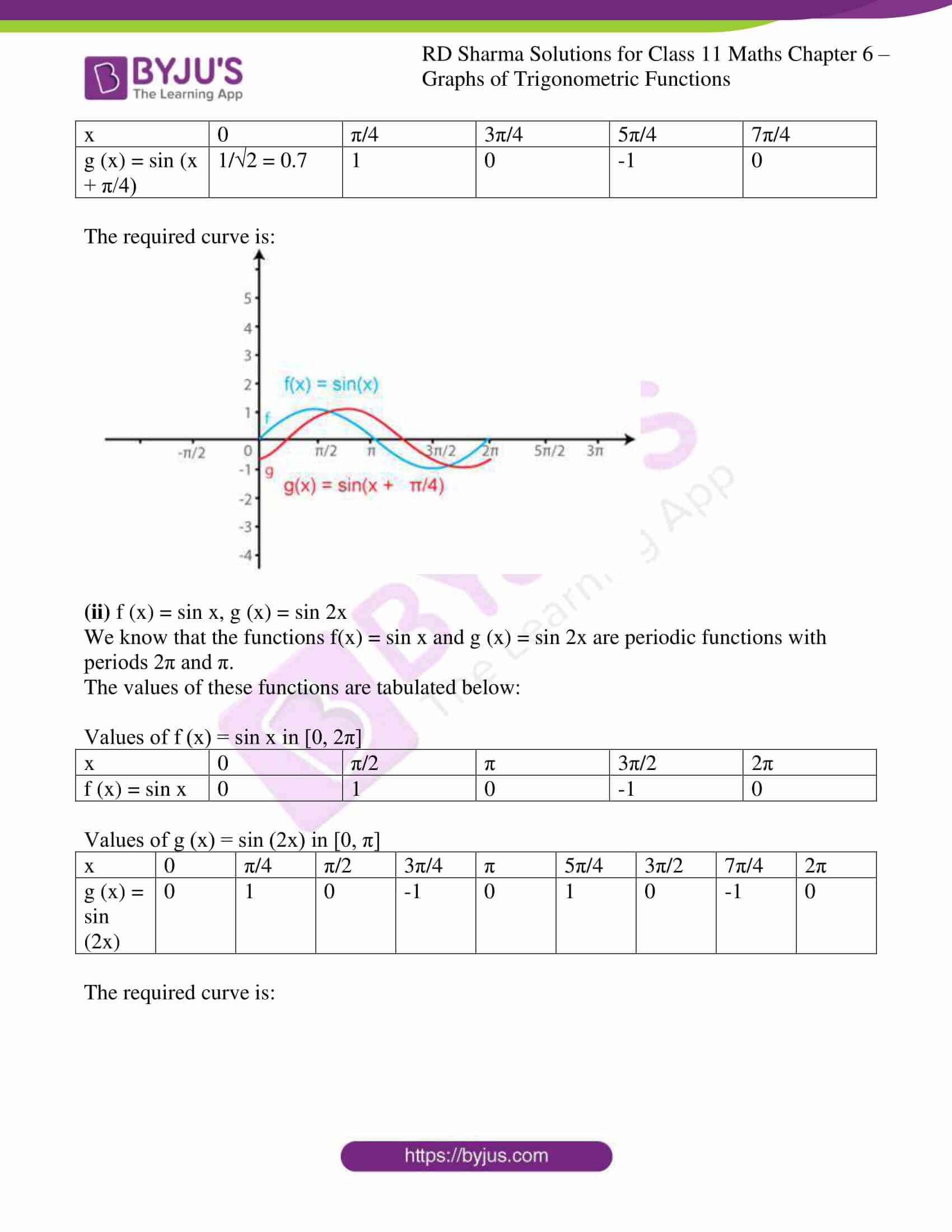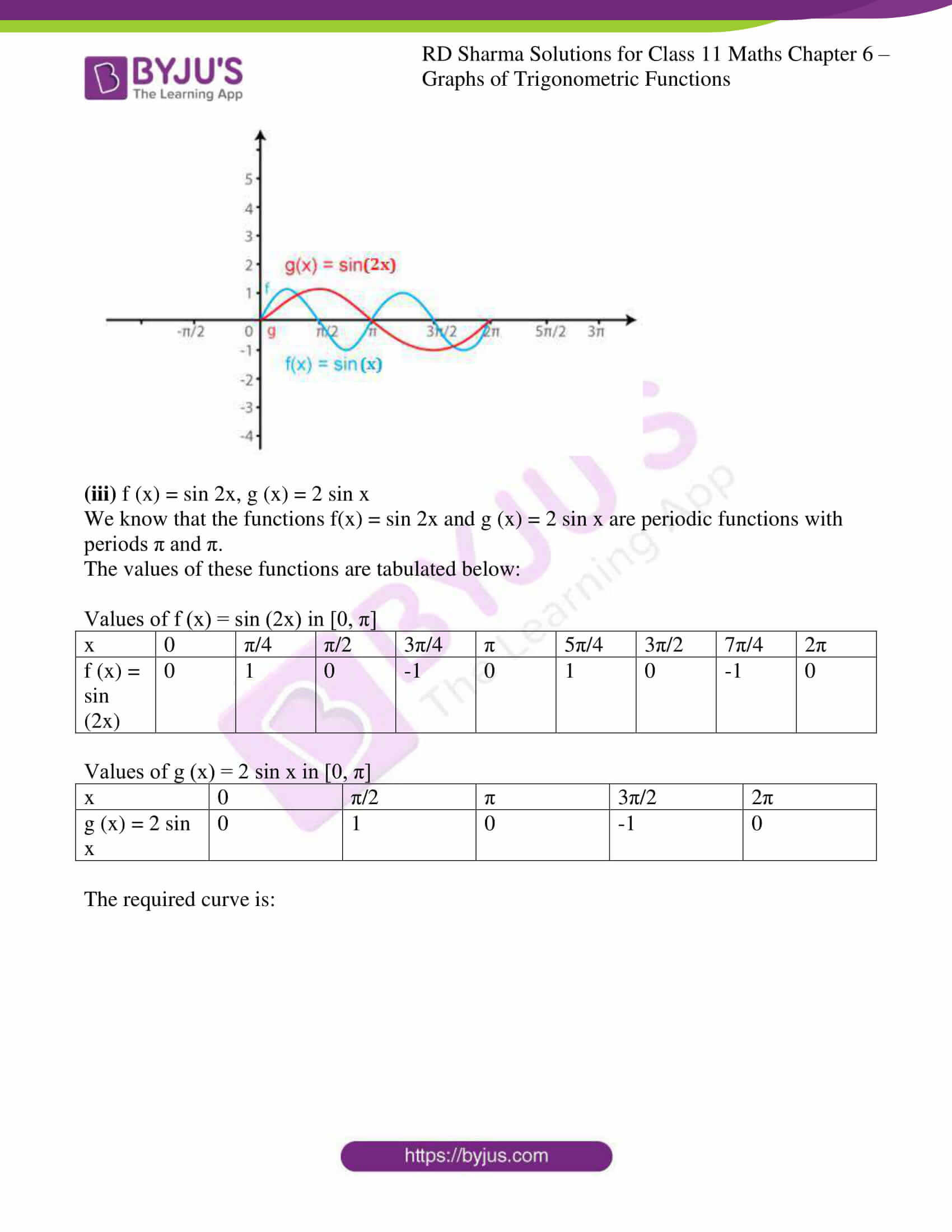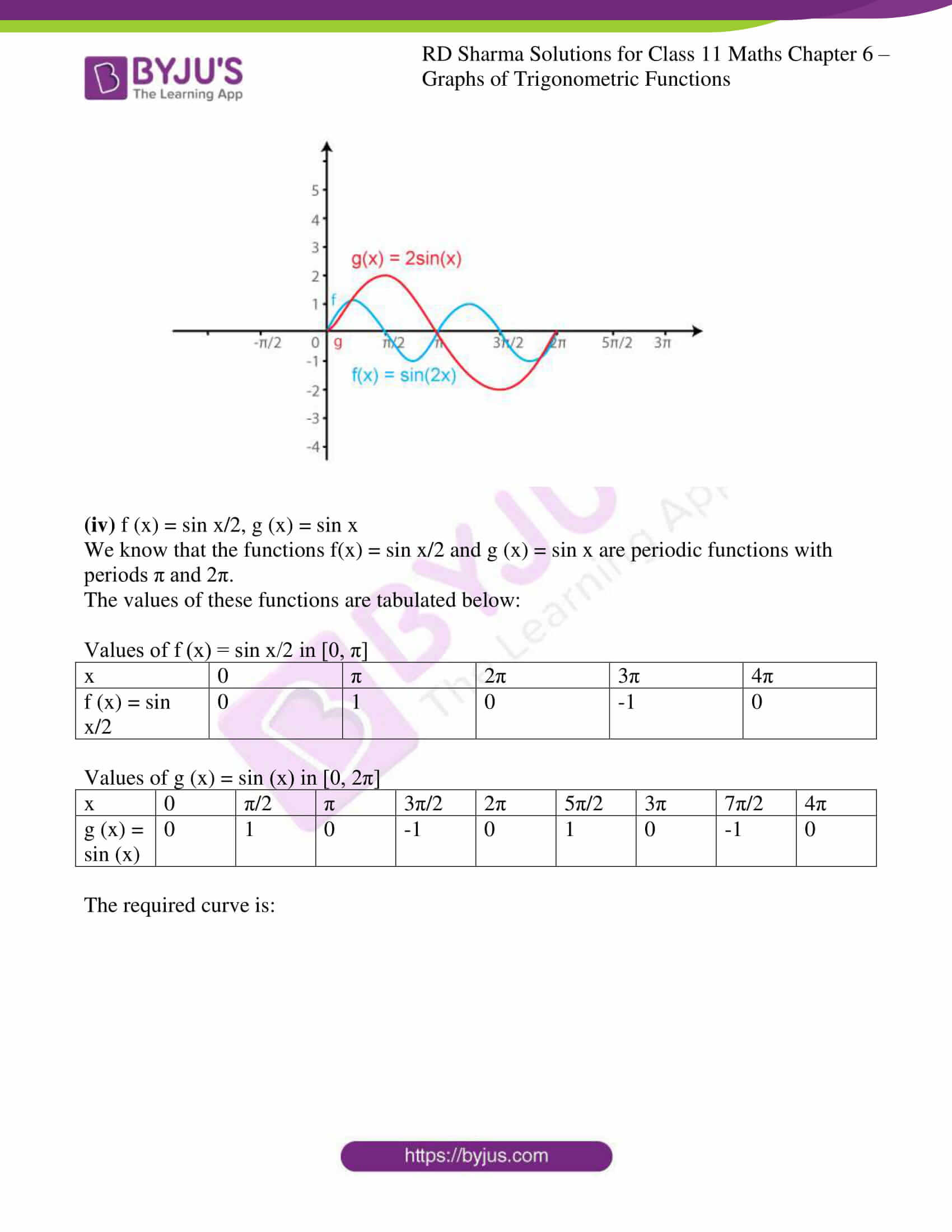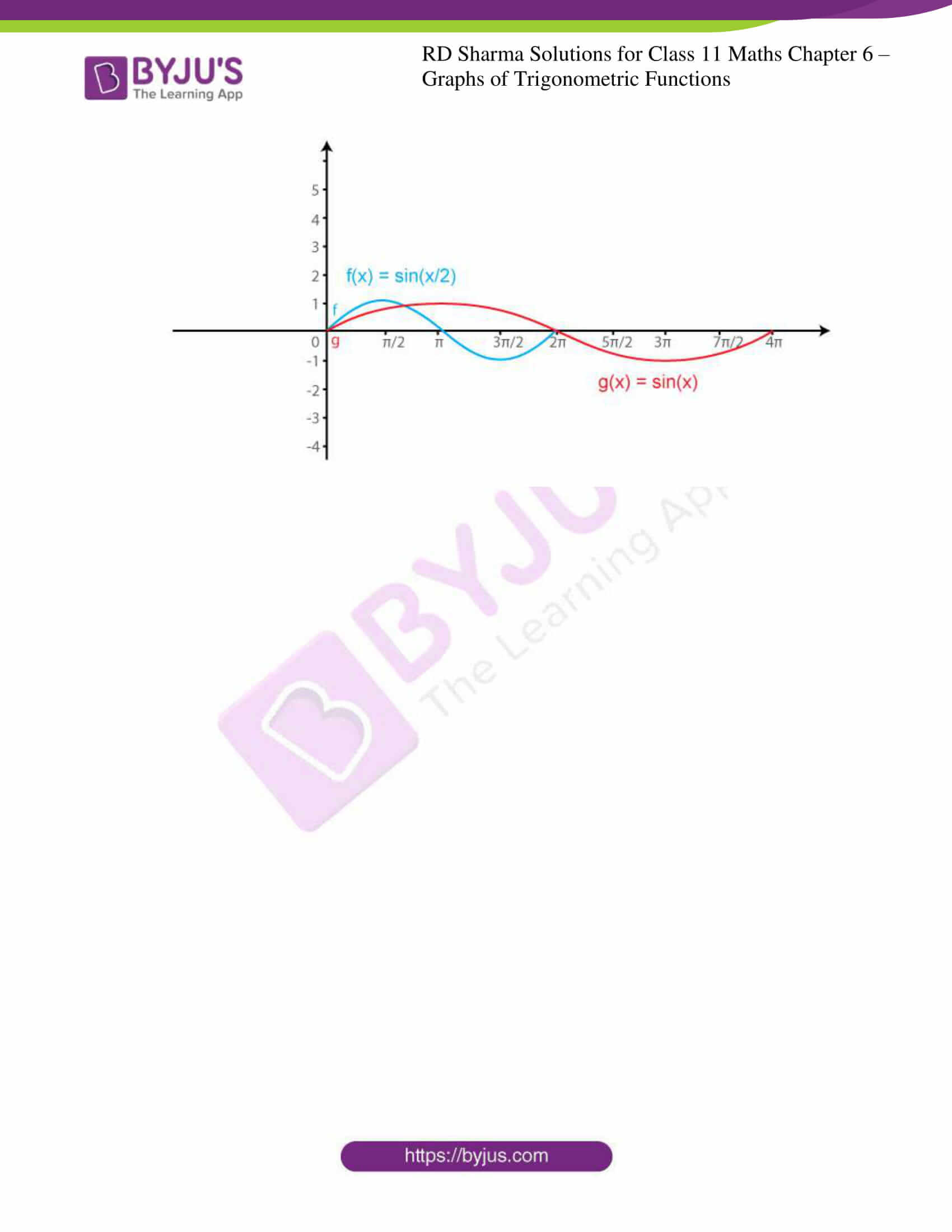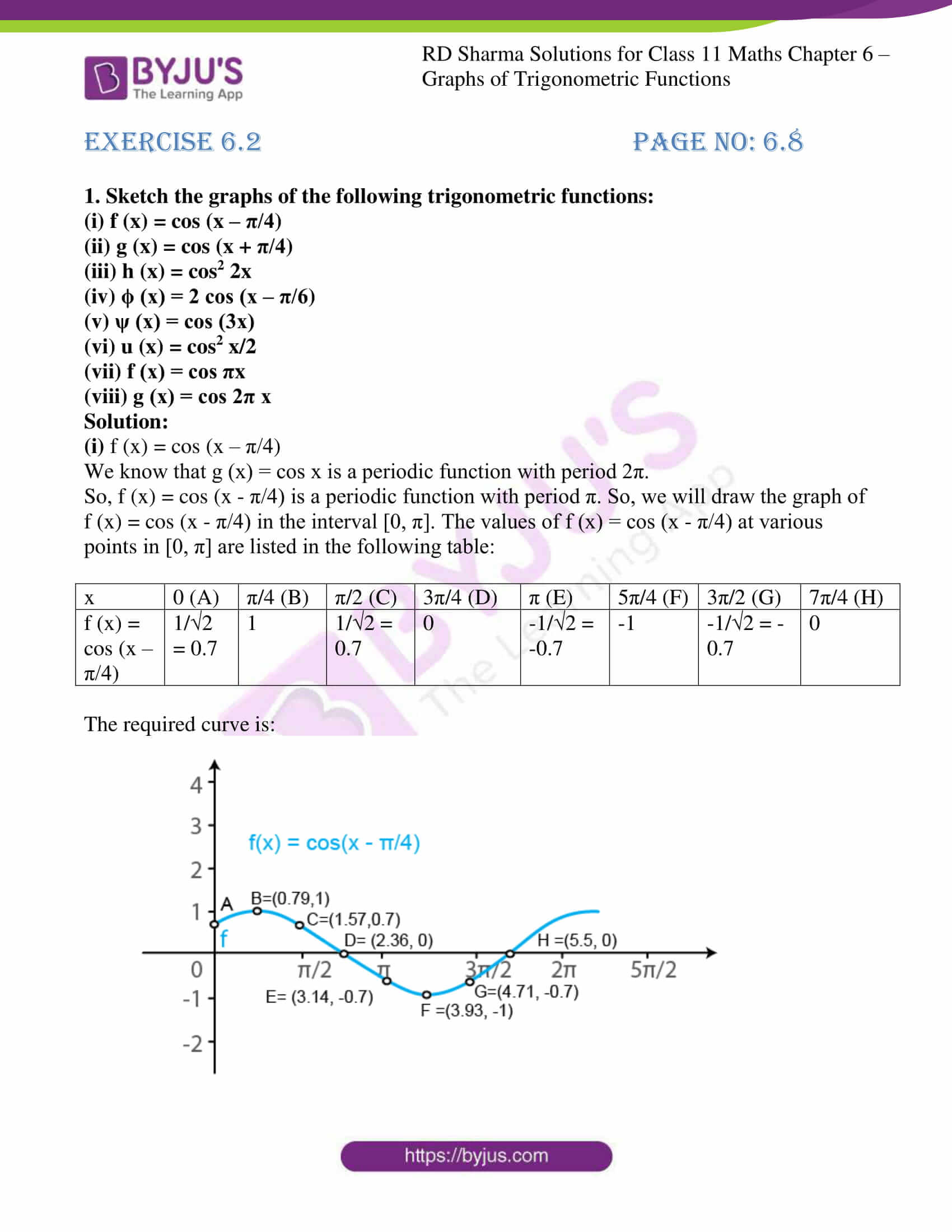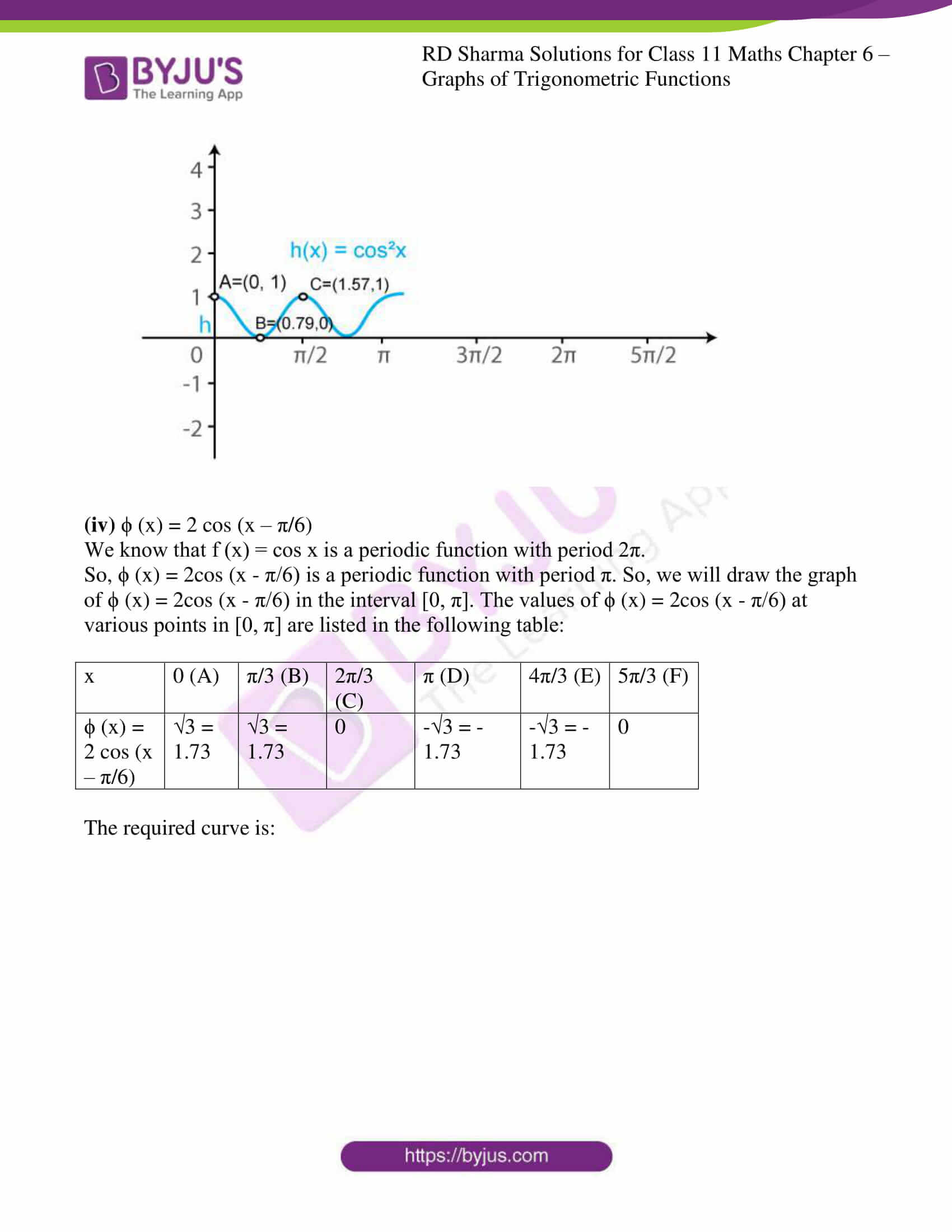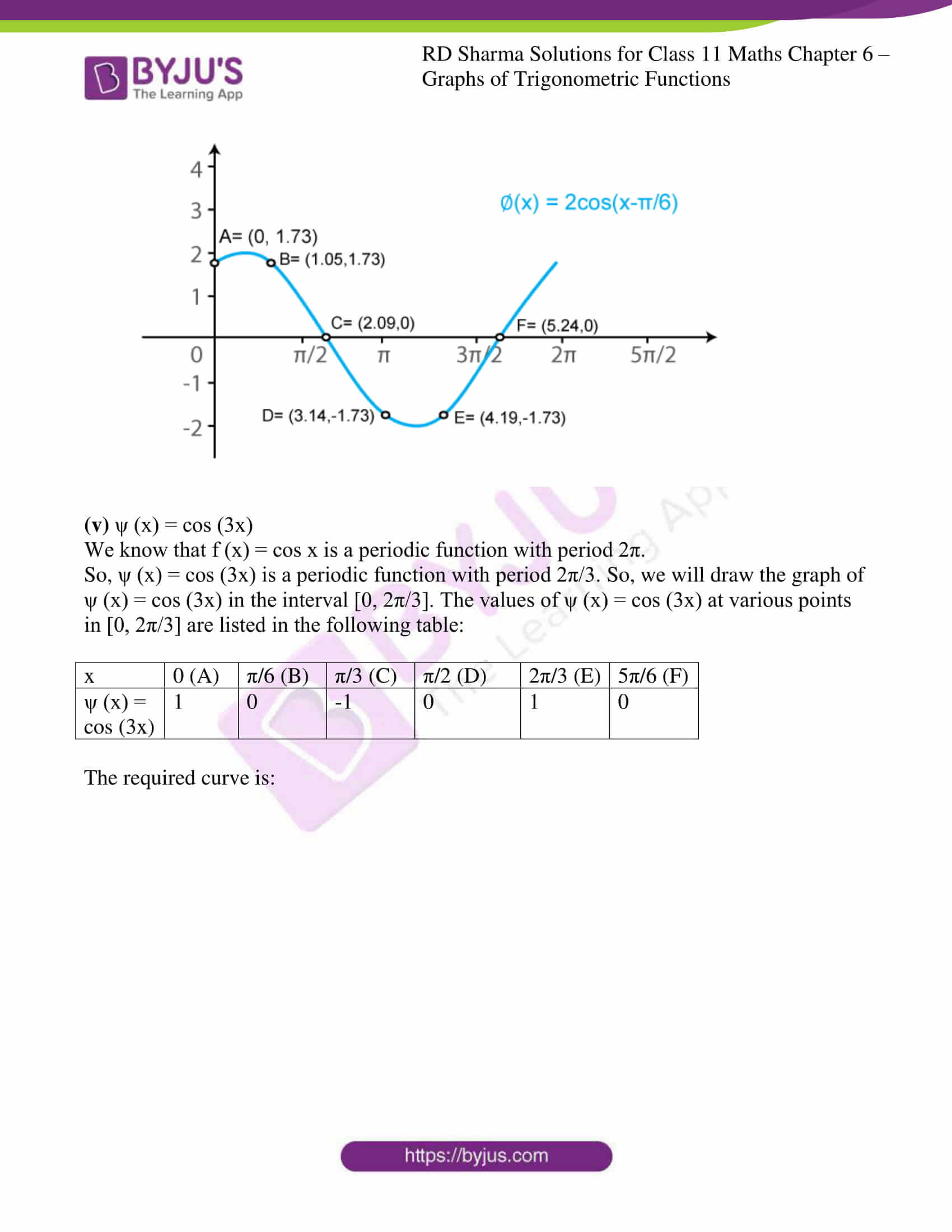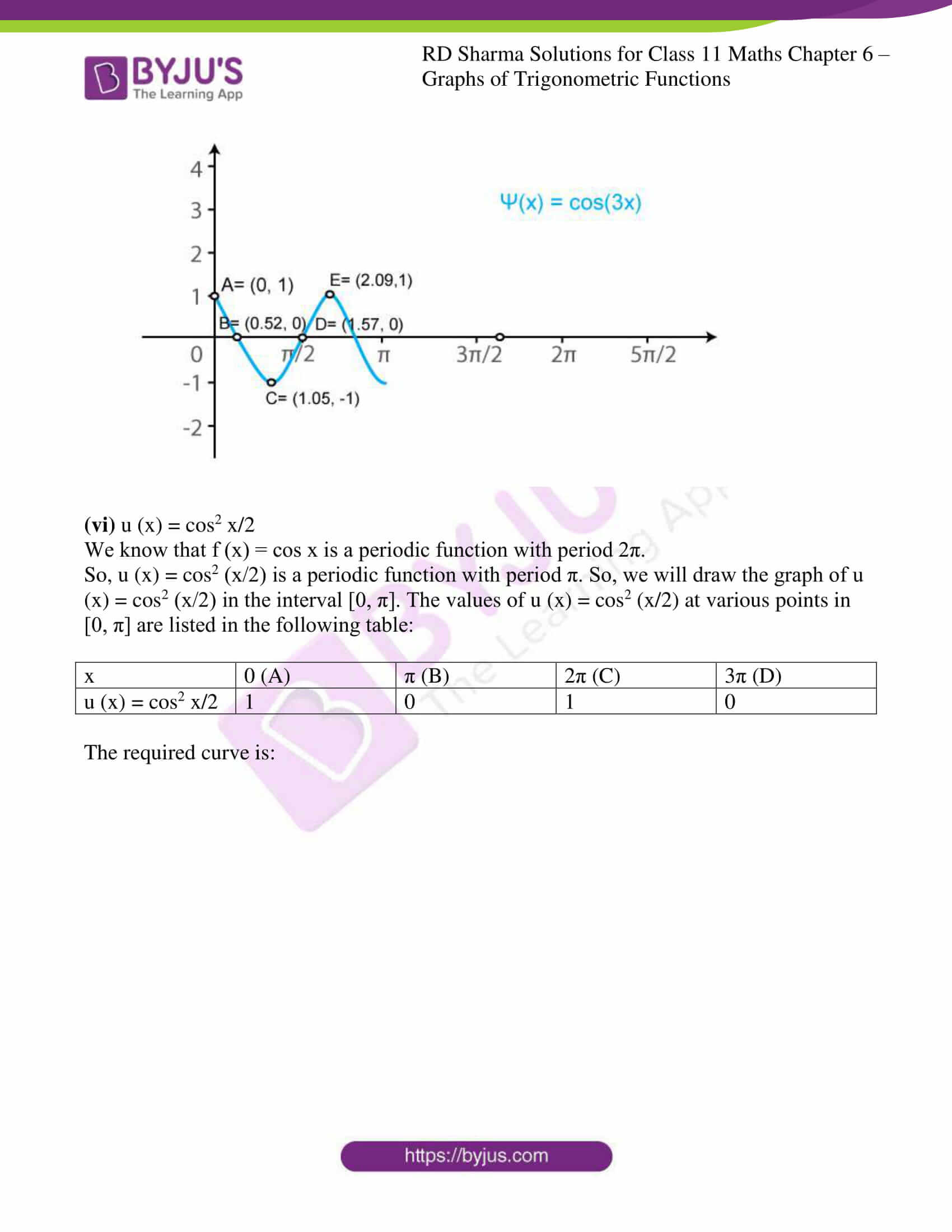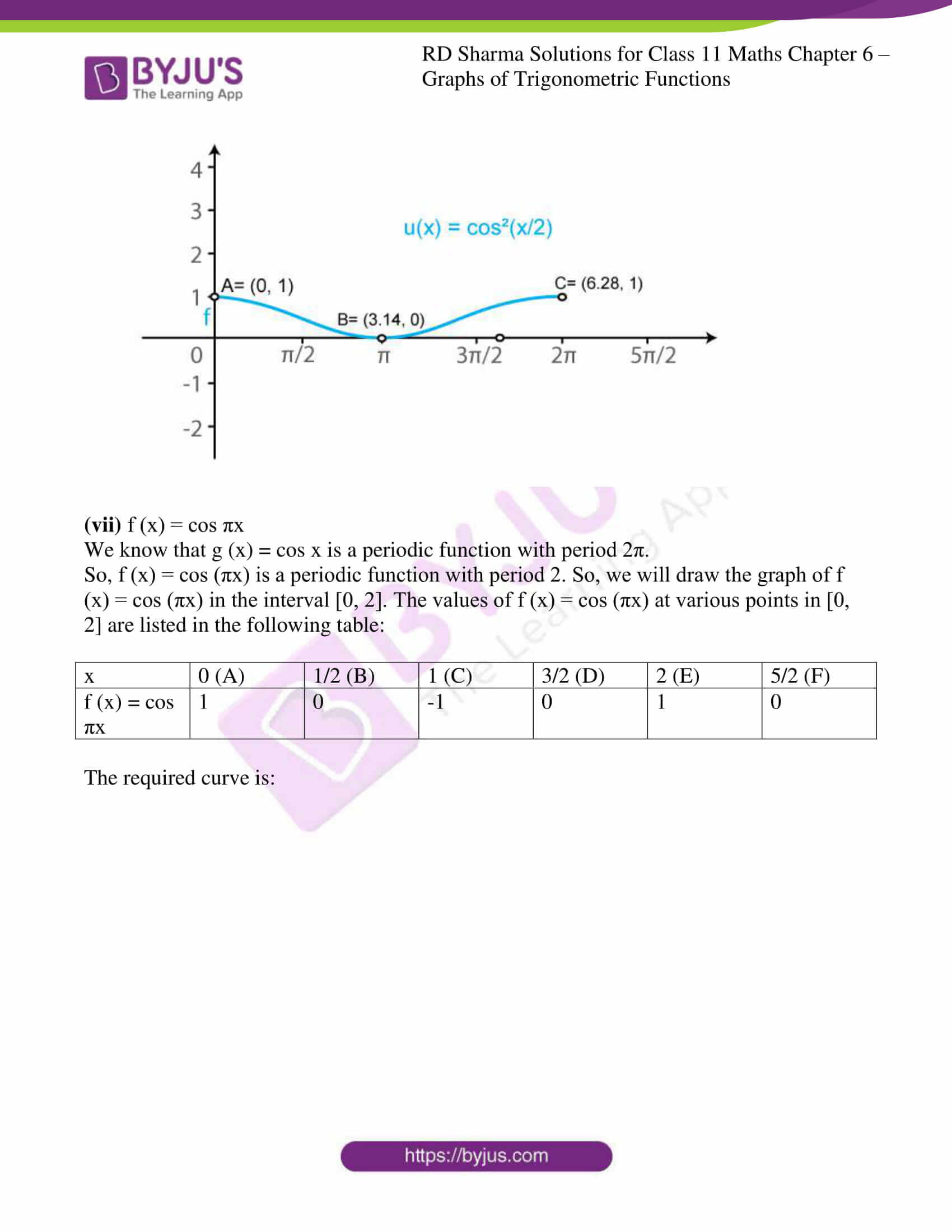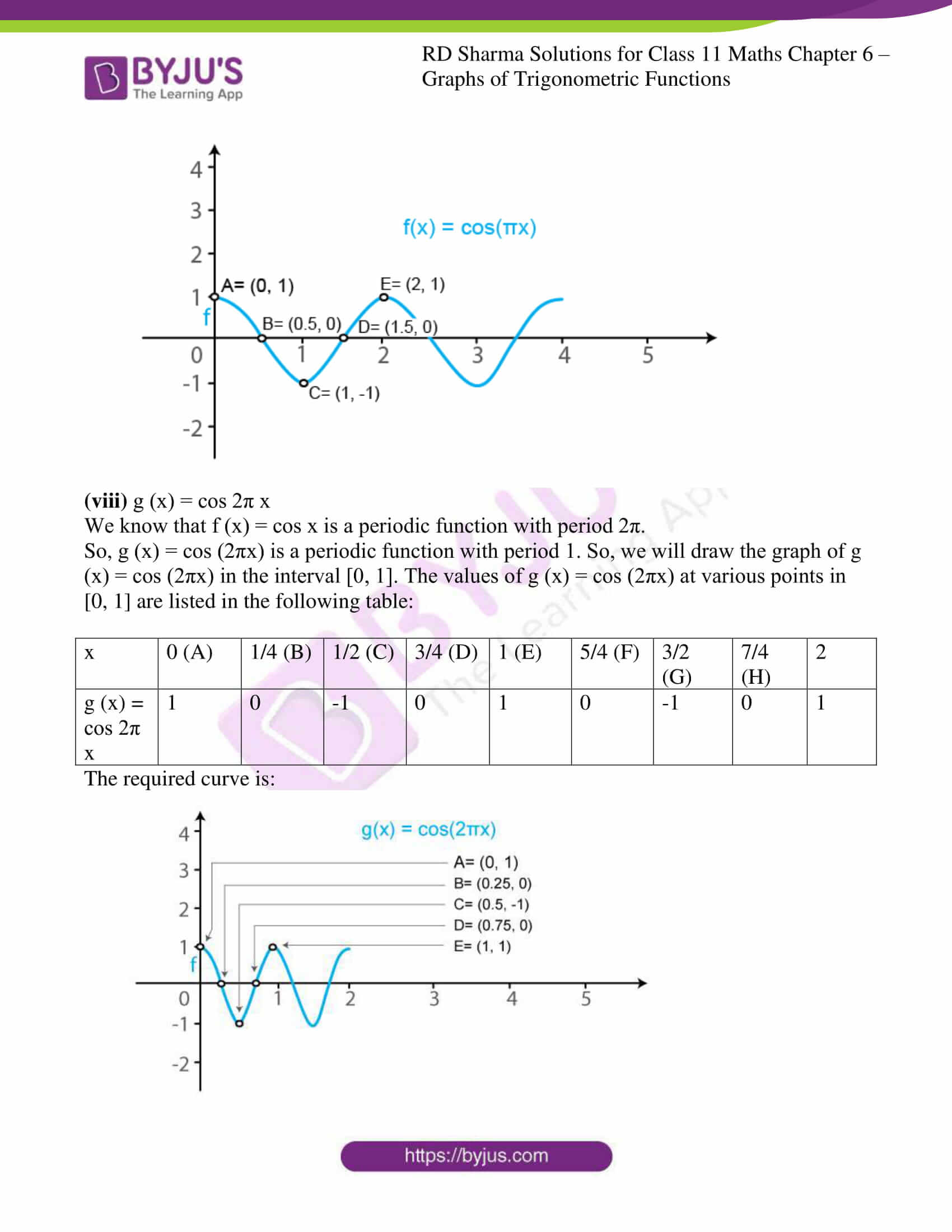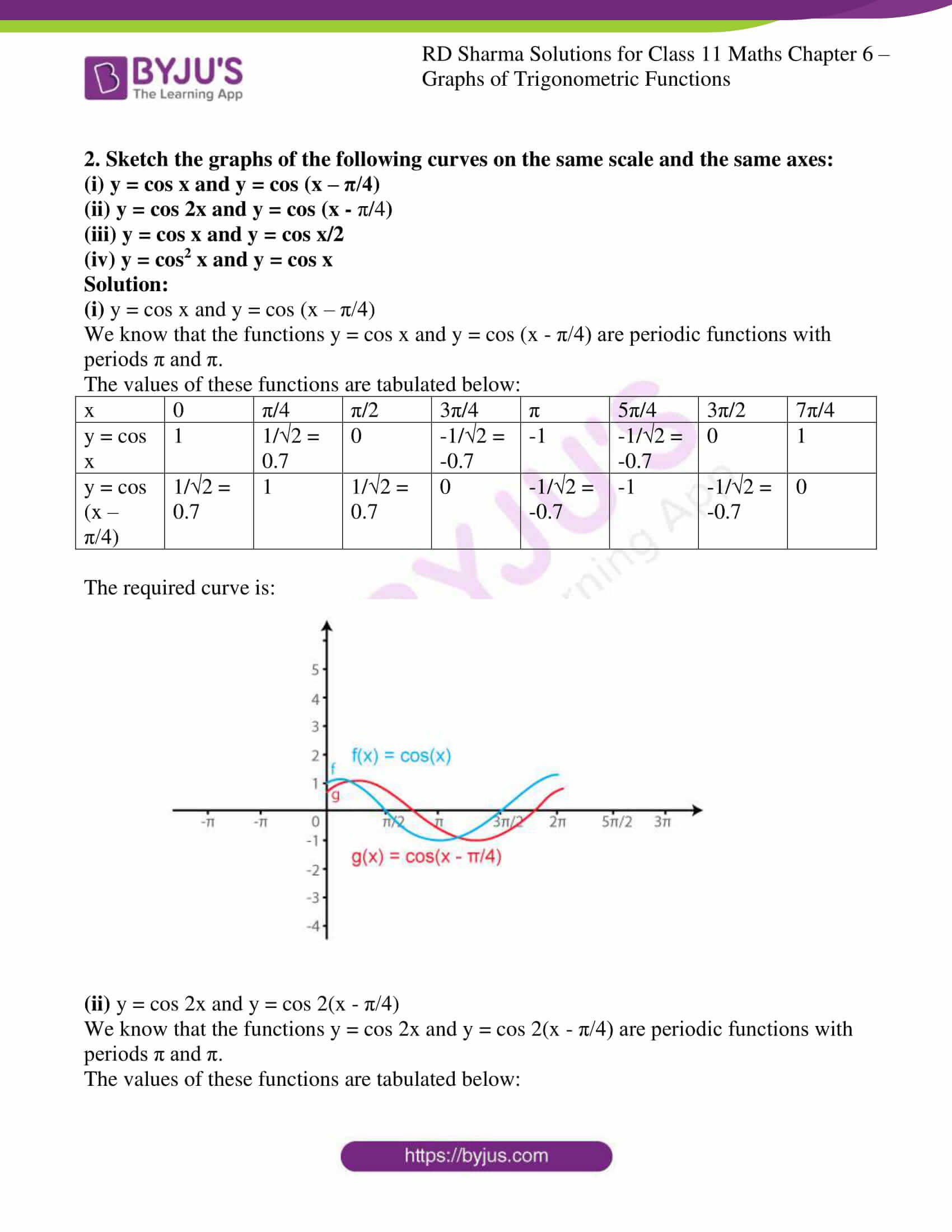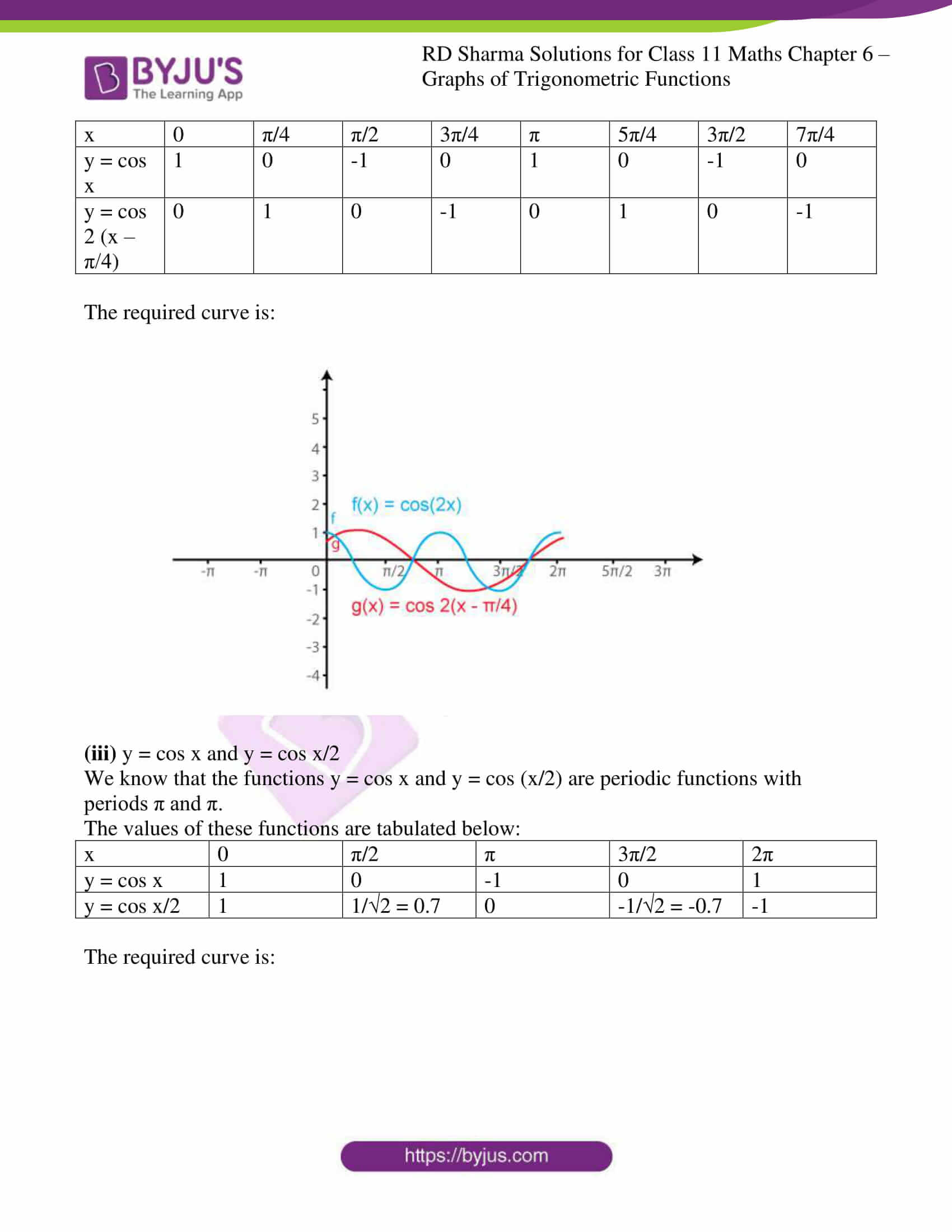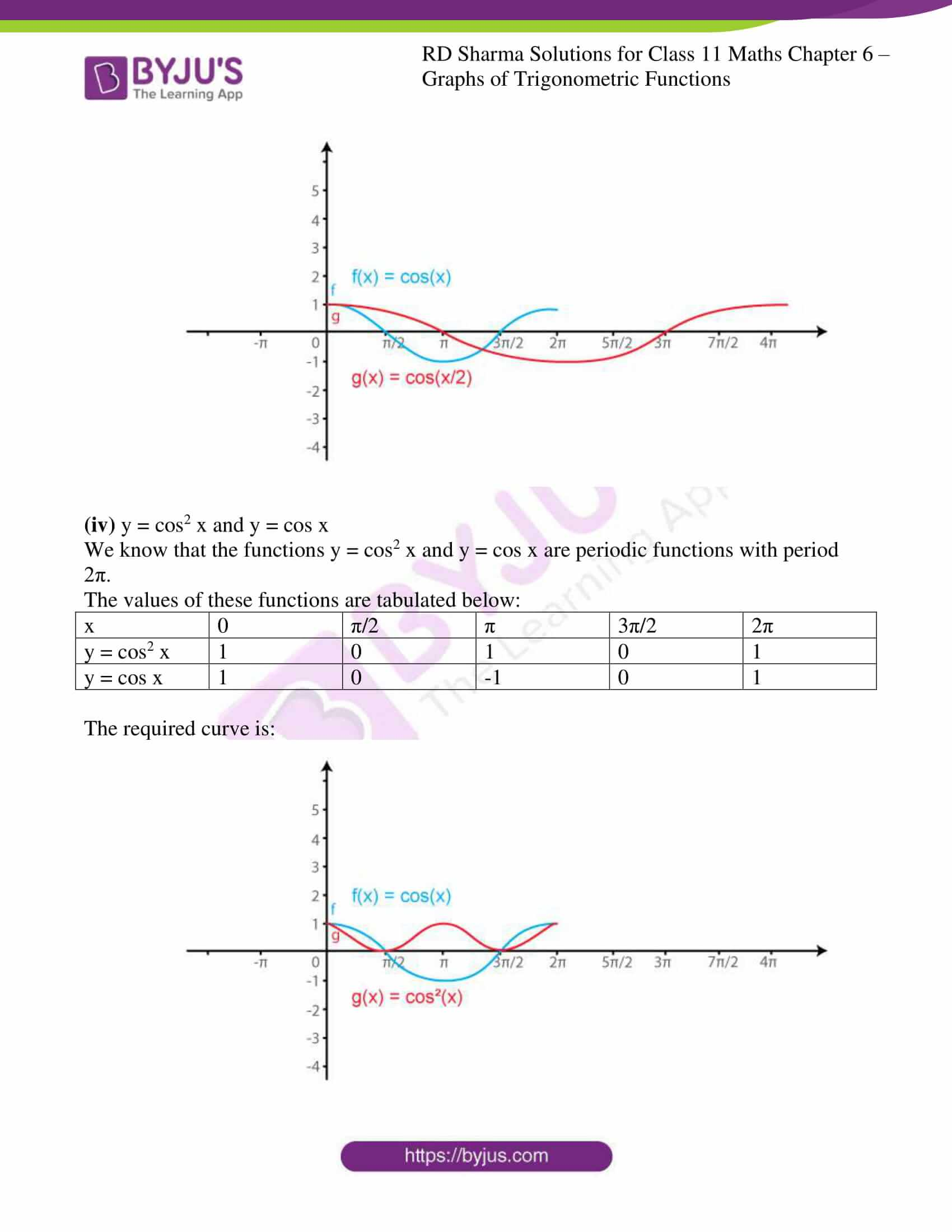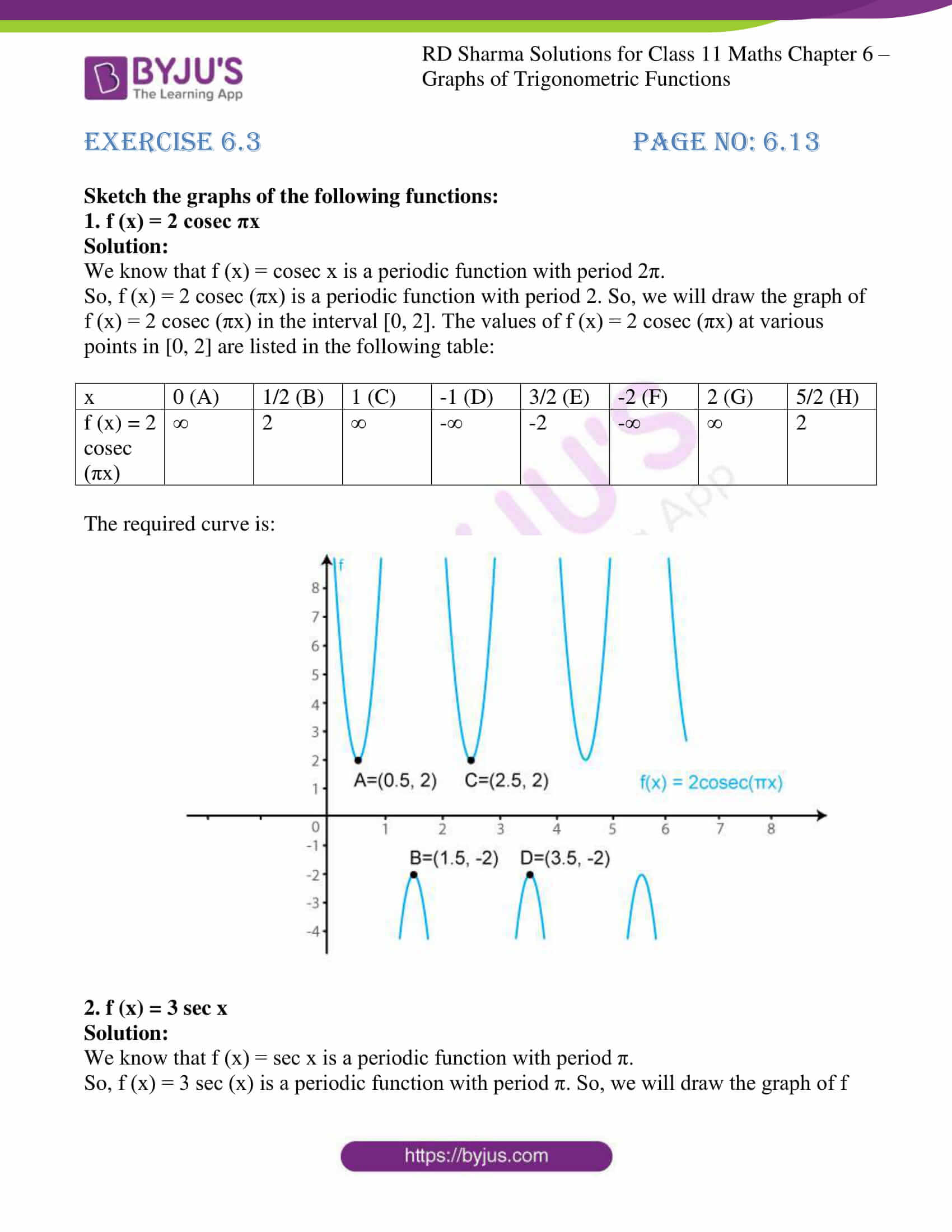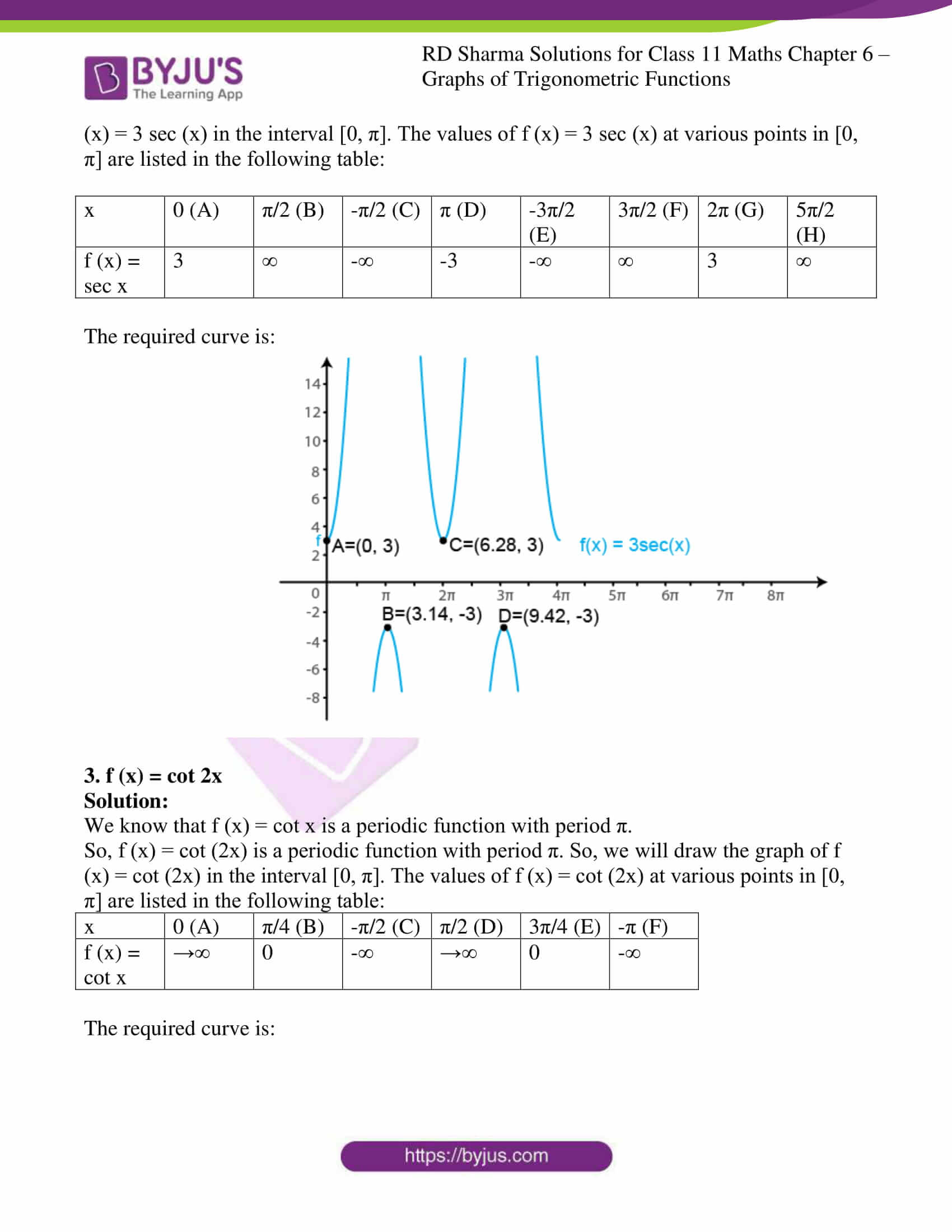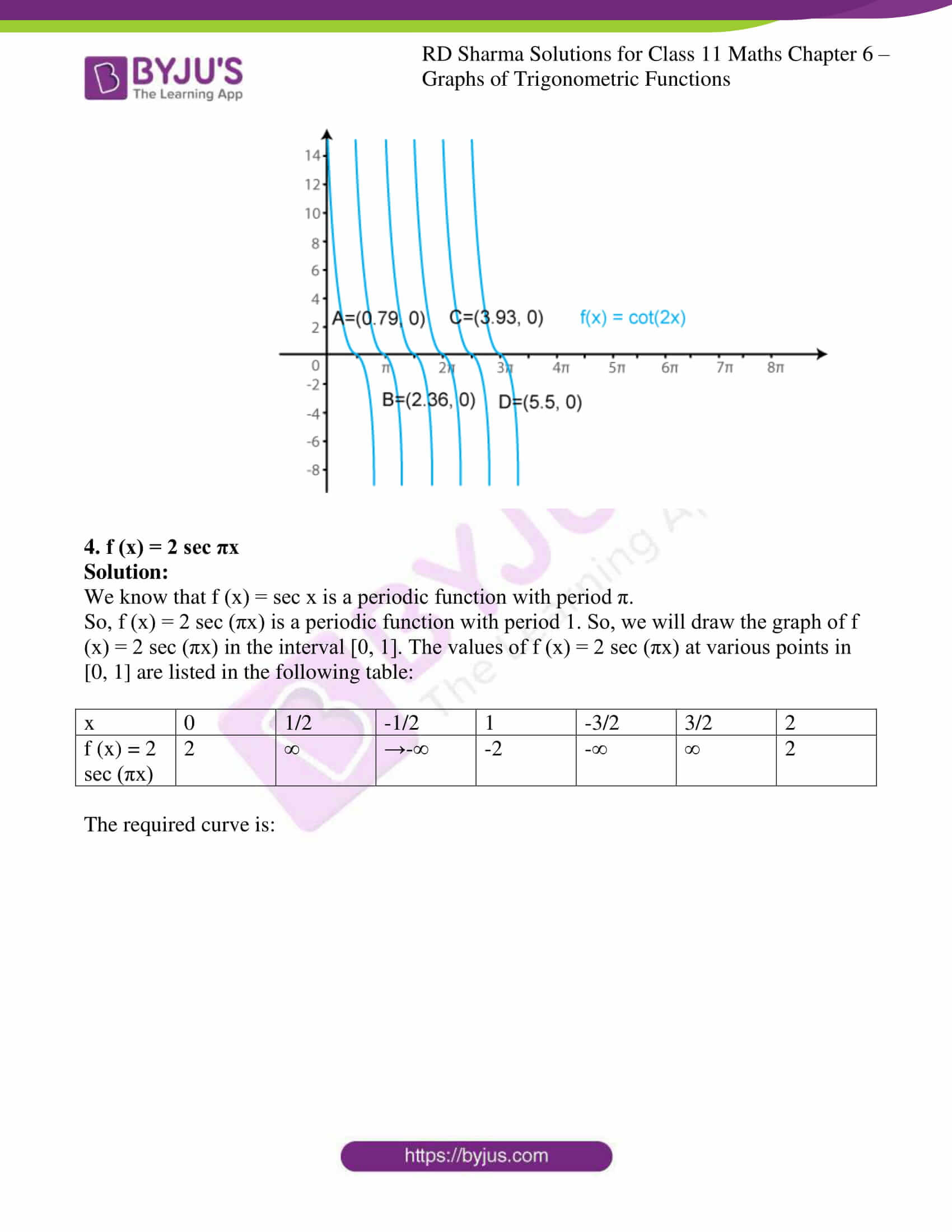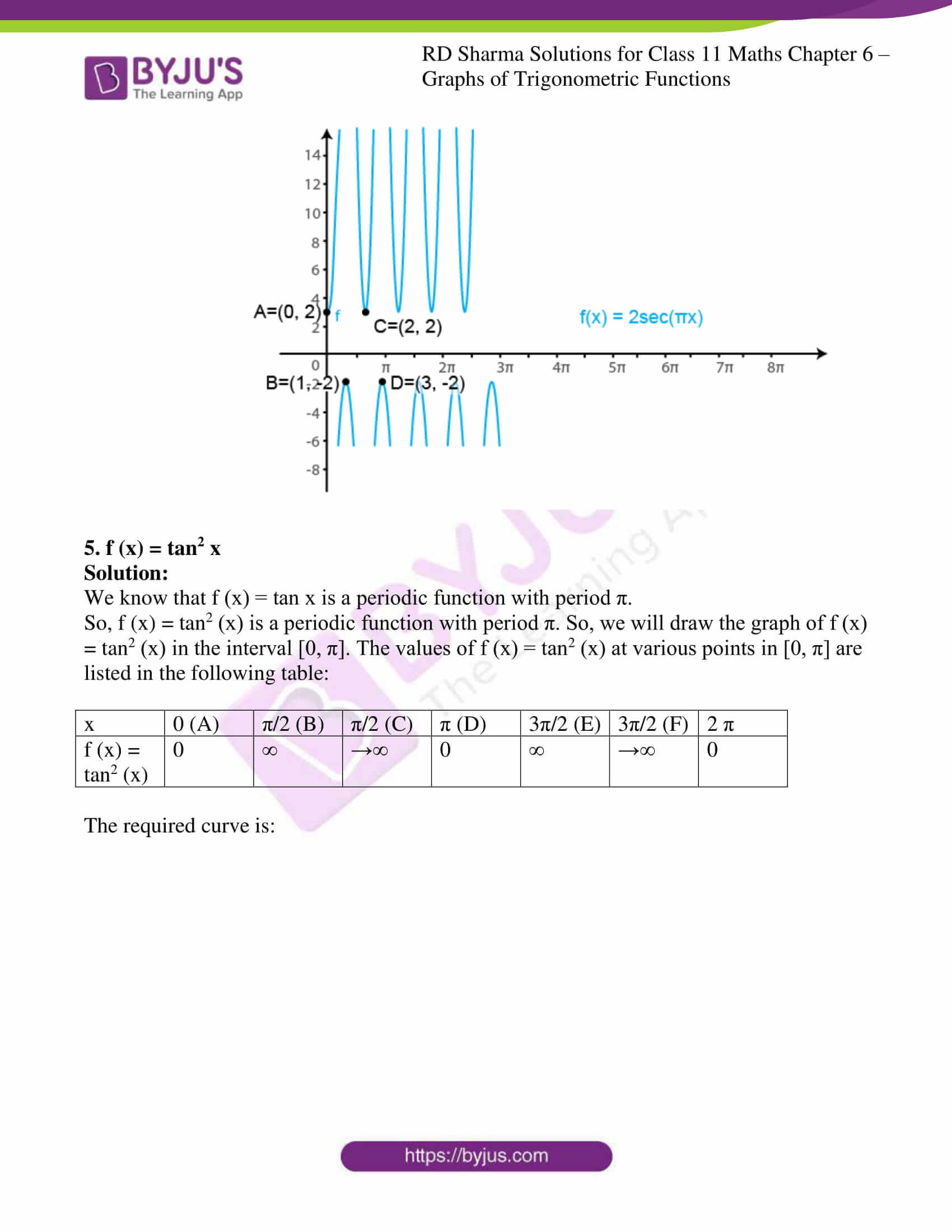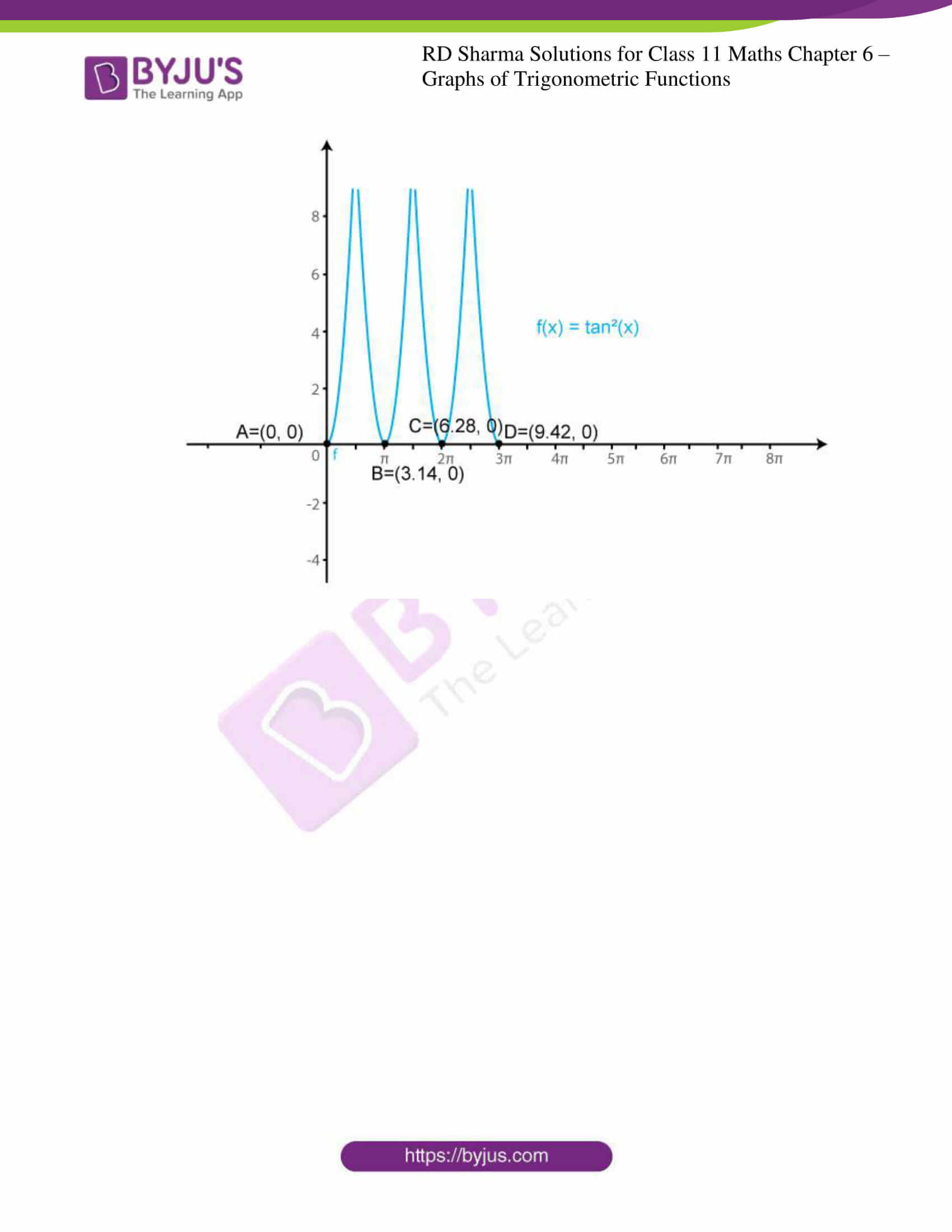### Also, access exercises of RD Sharma Solutions for Class 11 Maths Chapter 6 – Graphs of Trigonometric Functions

Exercise 6.1 Solutions

Exercise 6.2 Solutions

Exercise 6.3 Solutions

### Access answers to RD Sharma Solutions for Class 11 Maths Chapter 6 – Graphs of Trigonometric Functions

EXERCISE 6.1 PAGE NO: 6.5

1. Sketch the graphs of the following functions:

(i) f (x) = 2 sin x, 0 ≤ x ≤ π

(ii) g (x) = 3 sin (x – π/4), 0 ≤ x ≤ 5π/4

(iii) h (x) = 2 sin 3x, 0 ≤ x ≤ 2π/3

(iv) ϕ (x) = 2 sin (2x – π/3), 0 ≤ x ≤ 7π/3

(v) Ψ (x) = 4 sin 3 (x – π/4), 0 ≤ x ≤ 2π

(vi) θ (x) = sin (x/2 – π/4), 0 ≤ x ≤ 4π

(vii) u (x) = sin2 x, 0 ≤ x ≤ 2π υ (x) = |sin x|, 0 ≤ x ≤ 2π

(viii) f (x) = 2 sin πx, 0 ≤ x ≤ 2

Solution:

(i) f (x) = 2 sin x, 0 ≤ x ≤ π

We know that g (x) = sin x is a periodic function with period π.

So, f (x) = 2 sin x is a periodic function with period π. So, we will draw the graph of f (x) = 2 sin x in the interval [0, π]. The values of f (x) = 2 sin x at various points in [0, π] are listed in the following table:

 x 0(A) π/6 (B) π/3 (C) π/2 (D) 2π/3 (E) 5π/6 (F) Π (G) f (x) = 2 sin x 0 1 √3 = 1.73 2 √3 = 1.73 1 0

The required curve is: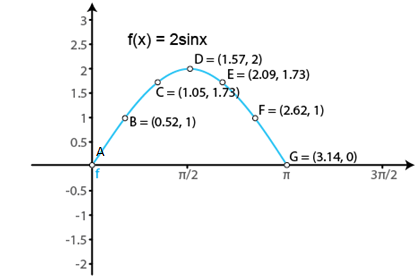(ii) g (x) = 3 sin (x – π/4), 0 ≤ x ≤ 5π/4

We know that if f (x) is a periodic function with period T, then f (ax + b) is periodic with period T/|a|.

So, g (x) = 3 sin (x – π/4) is a periodic function with period π. So, we will draw the graph of g (x) = 3 sin (x – π/4) in the interval [0, 5π/4]. The values of g (x) = 3 sin (x – π/4) at various points in [0, 5π/4] are listed in the following table:

 x 0(A) π/4 (B) π/2 (C) 3π/4 (D) π (E) 5π/4 (F) g (x) = 3 sin (x – π/4) -3/√2 = -2.1 0 3/√2 = 2.12 3 3/√2 = 2.12 0

The required curve is: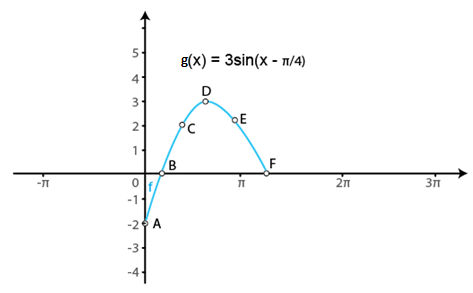(iii) h (x) = 2 sin 3x, 0 ≤ x ≤ 2π/3

We know that g (x) = sin x is a periodic function with period 2π.

So, h (x) = 2 sin 3x is a periodic function with period 2π/3. So, we will draw the graph of h (x) = 2 sin 3x in the interval [0, 2π/3]. The values of h (x) = 2 sin 3x at various points in [0, 2π/3] are listed in the following table:

 x 0 (A) π/6 (B) π/3 (C) π/2 (D) 2π/3 (E) h (x) = 2 sin 3x 0 2 0 -2 0

The required curve is: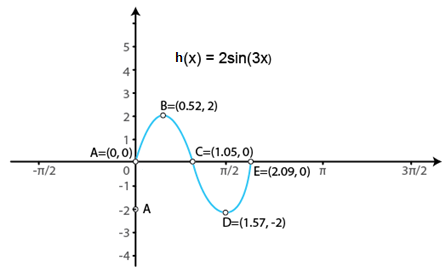(iv) ϕ (x) = 2 sin (2x – π/3), 0 ≤ x ≤ 7π/3

We know that if f(x) is a periodic function with period T, then f (ax + b) is periodic with period T/|a|.

So, ϕ (x) = 2 sin (2x – π/3) is a periodic function with period π. So, we will draw the graph of ϕ (x) = 2 sin (2x – π/3), in the interval [0, 7π/5]. The values of ϕ (x) = 2 sin (2x – π/3), at various points in [0, 7π/5] are listed in the following table:

 x 0 (A) π/6 (B) 2π/3 (C) 7π/6 (D) 7π/5 (E) ϕ (x) = 2 sin (2x – π/3) -√3 = -1.73 0 0 0 1.98

The required curve is: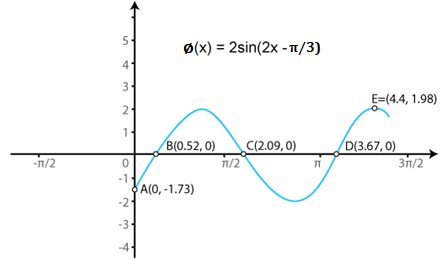(v) Ψ (x) = 4 sin 3 (x – π/4), 0 ≤ x ≤ 2π

We know that if f(x) is a periodic function with period T, then f (ax + b) is periodic with period T/|a|.

So, Ψ (x) = 4 sin 3 (x – π/4) is a periodic function with period 2π. So, we will draw the graph of Ψ (x) = 4 sin 3 (x – π/4) in the interval [0, 2π]. The values of Ψ (x) = 4 sin 3 (x – π/4) at various points in [0, 2π] are listed in the following table:

 x 0 (A) π/4 (B) π/2 (C) π (D) 5π/4 (E) 2π (F) Ψ (x) = 4 sin 3 (x – π/4) -2√2 = -2.82 0 2√2 = 2.82 0 1.98 -2√2 = -2.82

The required curve is: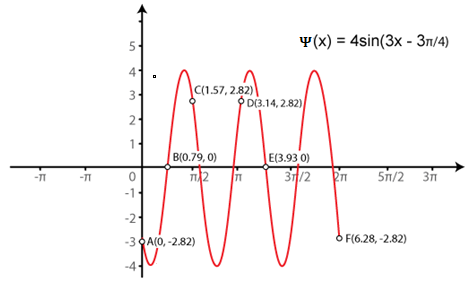(vi) θ (x) = sin (x/2 – π/4), 0 ≤ x ≤ 4π

We know that if f(x) is a periodic function with period T, then f (ax + b) is periodic with period T/|a|.

So, θ (x) = sin (x/2 – π/4) is a periodic function with period 4π. So, we will draw the graph of θ (x) = sin (x/2 – π/4) in the interval [0, 4π]. The values of θ (x) = sin (x/2 – π/4) at various points in [0, 4π] are listed in the following table:

 x 0 (A) π/2 (B) π (C) 2π (D) 5π/2 (E) 3π (F) 4π (G) θ (x) = sin (x/2 – π/4) -1/√2 = -0.7 0 1/√2 = 0.7 1/√2 = 0.7 0 -1/√2 = -0.7 -1/√2 = -0.7

The required curve is: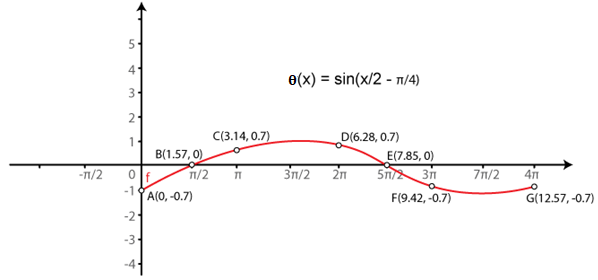(vii) u (x) = sin2 x, 0 ≤ x ≤ 2π υ (x) = |sin x|, 0 ≤ x ≤ 2π

We know that g (x) = sin x is a periodic function with period π.

So, u (x) = sin2 x is a periodic function with period 2π. So, we will draw the graph of u (x) = sin2 x in the interval [0, 2π]. The values of u (x) = sin2 x at various points in [0, 2π] are listed in the following table:

 x 0 (A) π/2 (B) Π (C) 3π/2 (D) 2π (E) u (x) = sin2 x 0 1 0 1 0

The required curve is: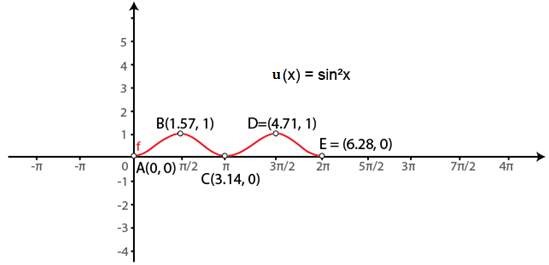(viii) f (x) = 2 sin πx, 0 ≤ x ≤ 2

We know that g (x) = sin x is a periodic function with period 2π.

So, f (x) = 2 sin πx is a periodic function with period 2. So, we will draw the graph of f (x) = 2 sin πx in the interval [0, 2]. The values of f (x) = 2 sin πx at various points in [0, 2] are listed in the following table:

 x 0 (A) 1/2 (B) 1 (C) 3/2 (D) 2 (E) f (x) = 2 sin πx 0 2 0 -2 0

The required curve is: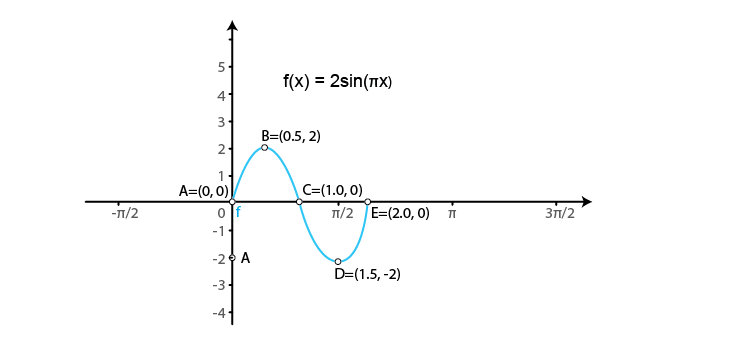2. Sketch the graphs of the following pairs of functions on the same axes:

(i) f (x) = sin x, g (x) = sin (x + π/4)

(ii) f (x) = sin x, g (x) = sin 2x

(iii) f (x) = sin 2x, g (x) = 2 sin x

(iv) f (x) = sin x/2, g (x) = sin x

Solution:

(i) f (x) = sin x, g (x) = sin (x + π/4)

We know that the functions f (x) = sin x and g (x) = sin (x + π/4) are periodic functions with periods 2π and 7π/4.

The values of these functions are tabulated below:

Values of f (x) = sin x in [0, 2π]

 x 0 π/2 π 3π/2 2π f (x) = sin x 0 1 0 -1 0

Values of g (x) = sin (x + π/4) in [0, 7π/4]

 x 0 π/4 3π/4 5π/4 7π/4 g (x) = sin (x + π/4) 1/√2 = 0.7 1 0 -1 0

The required curve is: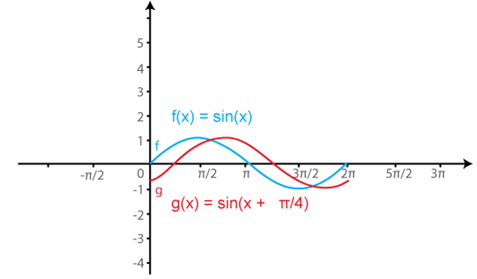(ii) f (x) = sin x, g (x) = sin 2x

We know that the functions f(x) = sin x and g (x) = sin 2x are periodic functions with periods 2π and π.

The values of these functions are tabulated below:

Values of f (x) = sin x in [0, 2π]

 x 0 π/2 π 3π/2 2π f (x) = sin x 0 1 0 -1 0

Values of g (x) = sin (2x) in [0, π]

 x 0 π/4 π/2 3π/4 π 5π/4 3π/2 7π/4 2π g (x) = sin (2x) 0 1 0 -1 0 1 0 -1 0

The required curve is: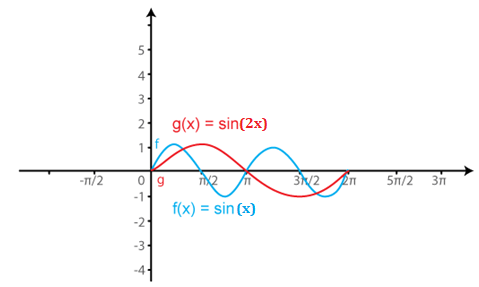(iii) f (x) = sin 2x, g (x) = 2 sin x

We know that the functions f(x) = sin 2x and g (x) = 2 sin x are periodic functions with periods π and π.

The values of these functions are tabulated below:

Values of f (x) = sin (2x) in [0, π]

 x 0 π/4 π/2 3π/4 π 5π/4 3π/2 7π/4 2π f (x) = sin (2x) 0 1 0 -1 0 1 0 -1 0

Values of g (x) = 2 sin x in [0, π]

 x 0 π/2 π 3π/2 2π g (x) = 2 sin x 0 1 0 -1 0

The required curve is: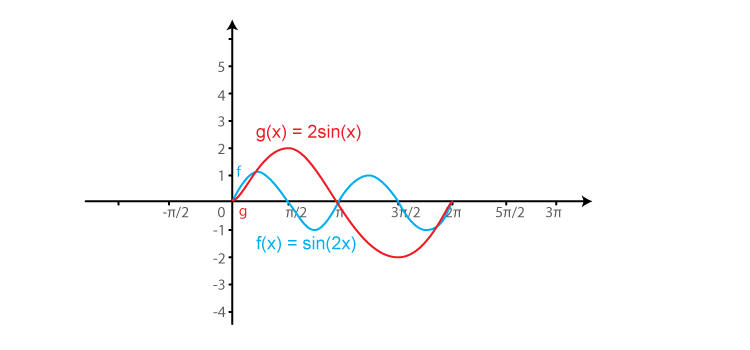(iv) f (x) = sin x/2, g (x) = sin x

We know that the functions f(x) = sin x/2 and g (x) = sin x are periodic functions with periods π and 2π.

The values of these functions are tabulated below:

Values of f (x) = sin x/2 in [0, π]

 x 0 π 2π 3π 4π f (x) = sin x/2 0 1 0 -1 0

Values of g (x) = sin (x) in [0, 2π]

 x 0 π/2 π 3π/2 2π 5π/2 3π 7π/2 4π g (x) = sin (x) 0 1 0 -1 0 1 0 -1 0

The required curve is: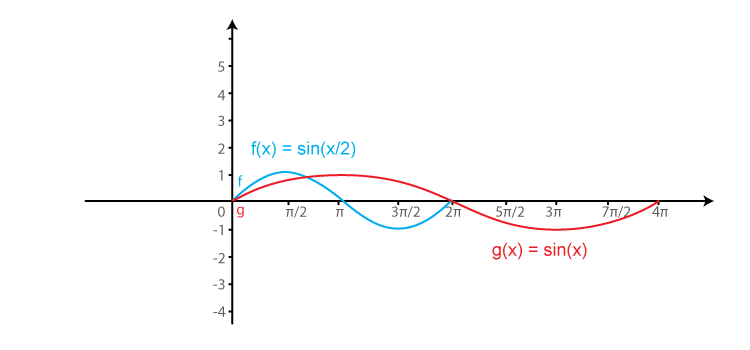EXERCISE 6.2 PAGE NO: 6.8

1. Sketch the graphs of the following trigonometric functions:

(i) f (x) = cos (x – π/4)

(ii) g (x) = cos (x + π/4)

(iii) h (x) = cos2 2x

(iv) ϕ (x) = 2 cos (x – π/6)

(v) ψ (x) = cos (3x)

(vi) u (x) = cos2 x/2

(vii) f (x) = cos πx

(viii) g (x) = cos 2π x

Solution:

(i) f (x) = cos (x – π/4)

We know that g (x) = cos x is a periodic function with period 2π.

So, f (x) = cos (x – π/4) is a periodic function with period π. So, we will draw the graph of f (x) = cos (x – π/4) in the interval [0, π]. The values of f (x) = cos (x – π/4) at various points in [0, π] are listed in the following table:

 x 0 (A) π/4 (B) π/2 (C) 3π/4 (D) π (E) 5π/4 (F) 3π/2 (G) 7π/4 (H) f (x) = cos (x – π/4) 1/√2 = 0.7 1 1/√2 = 0.7 0 -1/√2 = -0.7 -1 -1/√2 = -0.7 0

The required curve is: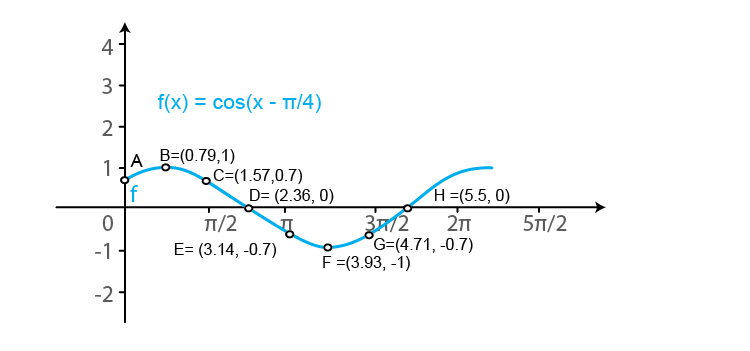(ii) g (x) = cos (x + π/4)

We know that f (x) = cos x is a periodic function with period 2π.

So, g (x) = cos (x + π/4) is a periodic function with period π. So, we will draw the graph of g (x) = cos (x + π/4) in the interval [0, π]. The values of g (x) = cos (x + π/4) at various points in [0, π] are listed in the following table:

 x 0 (A) π/4 (B) π/2 (C) 3π/4 (D) π (E) 5π/4 (F) 3π/2 (G) 7π/4 (H) g (x) = cos (x + π/4) 1/√2 = 0.7 0 -1/√2 = -0.7 -1 -1/√2 = -0.7 0 1/√2 = 0.7 1

The required curve is: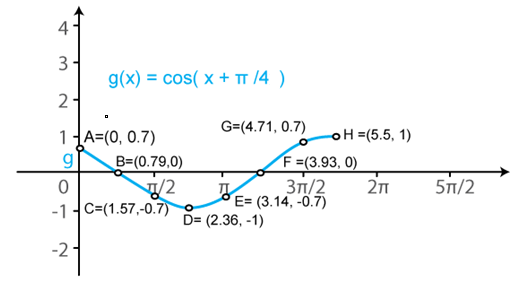(iii) h (x) = cos2 2x

We know that f (x) = cos x is a periodic function with period 2π.

So, h (x) = cos2 2x is a periodic function with period π. So, we will draw the graph of h (x) = cos2 2x in the interval [0, π]. The values of h (x) = cos2 2x at various points in [0, π] are listed in the following table:

 x 0 (A) π/4 (B) π/2 (C) 3π/4 (D) π (E) 5π/4 (F) 3π/2 (G) h (x) = cos2 2x 1 0 1 0 1 0 1

The required curve is: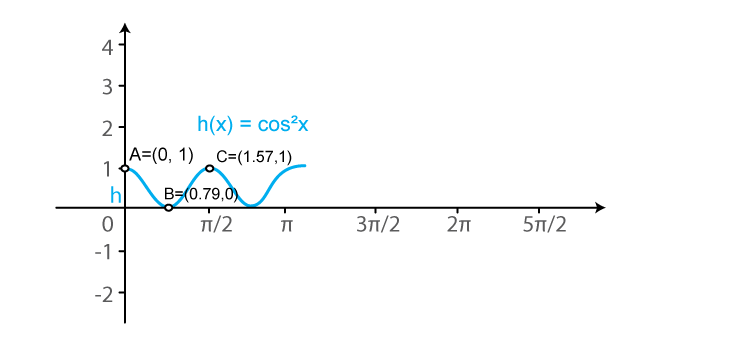(iv) ϕ (x) = 2 cos (x – π/6)

We know that f (x) = cos x is a periodic function with period 2π.

So, ϕ (x) = 2cos (x – π/6) is a periodic function with period π. So, we will draw the graph of ϕ (x) = 2cos (x – π/6) in the interval [0, π]. The values of ϕ (x) = 2cos (x – π/6) at various points in [0, π] are listed in the following table:

 x 0 (A) π/3 (B) 2π/3 (C) π (D) 4π/3 (E) 5π/3 (F) ϕ (x) = 2 cos (x – π/6) √3 = 1.73 √3 = 1.73 0 -√3 = -1.73 -√3 = -1.73 0

The required curve is: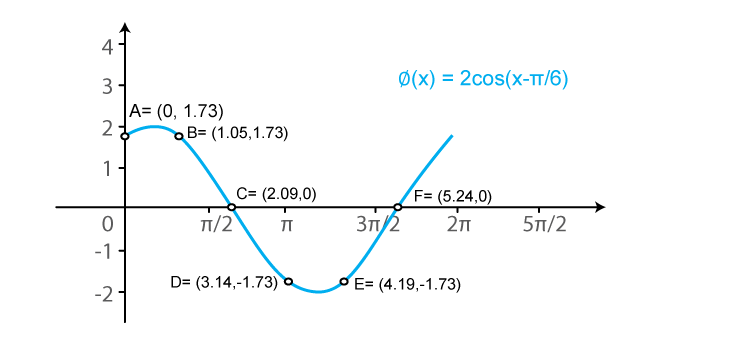(v) ψ (x) = cos (3x)

We know that f (x) = cos x is a periodic function with period 2π.

So, ψ (x) = cos (3x) is a periodic function with period 2π/3. So, we will draw the graph of ψ (x) = cos (3x) in the interval [0, 2π/3]. The values of ψ (x) = cos (3x) at various points in [0, 2π/3] are listed in the following table:

 x 0 (A) π/6 (B) π/3 (C) π/2 (D) 2π/3 (E) 5π/6 (F) ψ (x) = cos (3x) 1 0 -1 0 1 0

The required curve is: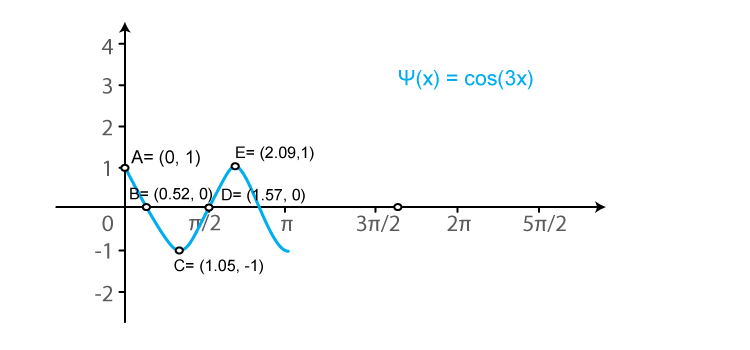(vi) u (x) = cos2 x/2

We know that f (x) = cos x is a periodic function with period 2π.

So, u (x) = cos2 (x/2) is a periodic function with period π. So, we will draw the graph of u (x) = cos2 (x/2) in the interval [0, π]. The values of u (x) = cos2 (x/2) at various points in [0, π] are listed in the following table:

 x 0 (A) π (B) 2π (C) 3π (D) u (x) = cos2 x/2 1 0 1 0

The required curve is: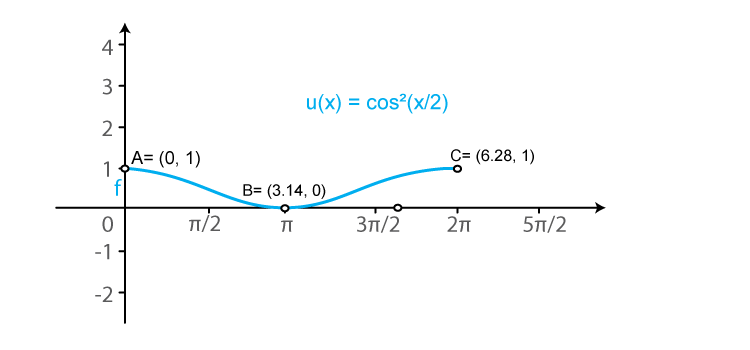(vii) f (x) = cos πx

We know that g (x) = cos x is a periodic function with period 2π.

So, f (x) = cos (πx) is a periodic function with period 2. So, we will draw the graph of f (x) = cos (πx) in the interval [0, 2]. The values of f (x) = cos (πx) at various points in [0, 2] are listed in the following table:

 x 0 (A) 1/2 (B) 1 (C) 3/2 (D) 2 (E) 5/2 (F) f (x) = cos πx 1 0 -1 0 1 0

The required curve is: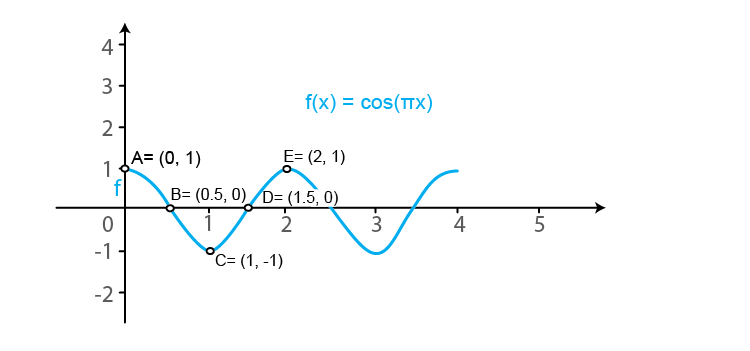(viii) g (x) = cos 2π x

We know that f (x) = cos x is a periodic function with period 2π.

So, g (x) = cos (2πx) is a periodic function with period 1. So, we will draw the graph of g (x) = cos (2πx) in the interval [0, 1]. The values of g (x) = cos (2πx) at various points in [0, 1] are listed in the following table:

 x 0 (A) 1/4 (B) 1/2 (C) 3/4 (D) 1 (E) 5/4 (F) 3/2 (G) 7/4 (H) 2 g (x) = cos 2π x 1 0 -1 0 1 0 -1 0 1

The required curve is: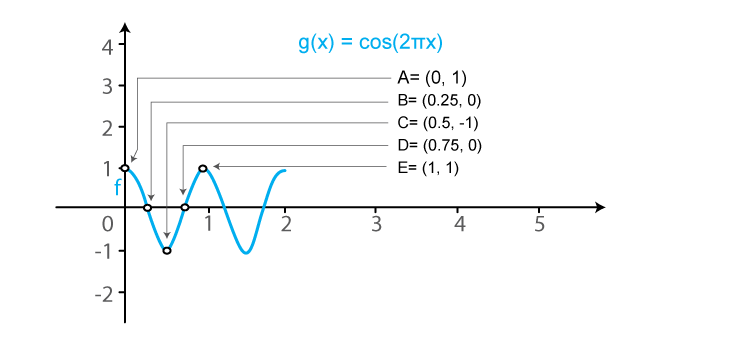2. Sketch the graphs of the following curves on the same scale and the same axes:

(i) y = cos x and y = cos (x – π/4)

(ii) y = cos 2x and y = cos (x – π/4

(iii) y = cos x and y = cos x/2

(iv) y = cos2 x and y = cos x

Solution:

(i) y = cos x and y = cos (x – π/4)

We know that the functions y = cos x and y = cos (x – π/4) are periodic functions with periods π and π.

The values of these functions are tabulated below:

 x 0 π/4 π/2 3π/4 π 5π/4 3π/2 7π/4 y = cos x 1 1/√2 = 0.7 0 -1/√2 = -0.7 -1 -1/√2 = -0.7 0 1 y = cos (x – π/4) 1/√2 = 0.7 1 1/√2 = 0.7 0 -1/√2 = -0.7 -1 -1/√2 = -0.7 0

The required curve is: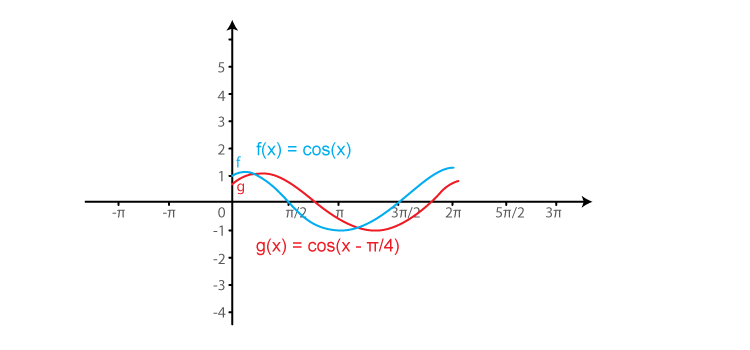(ii) y = cos 2x and y = cos 2(x – π/4)

We know that the functions y = cos 2x and y = cos 2(x – π/4) are periodic functions with periods π and π.

The values of these functions are tabulated below:

 x 0 π/4 π/2 3π/4 π 5π/4 3π/2 7π/4 y = cos x 1 0 -1 0 1 0 -1 0 y = cos 2 (x – π/4) 0 1 0 -1 0 1 0 -1

The required curve is: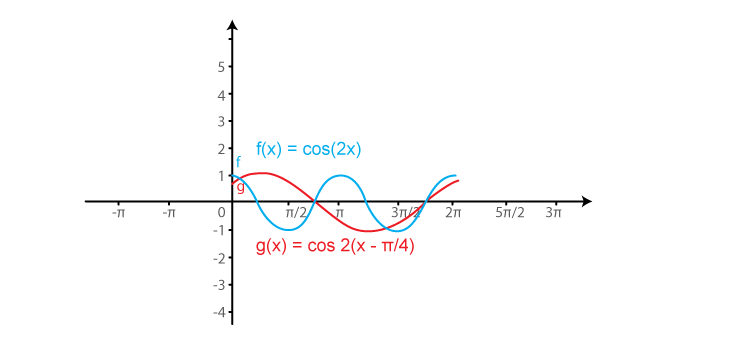(iii) y = cos x and y = cos x/2

We know that the functions y = cos x and y = cos (x/2) are periodic functions with periods π and π.

The values of these functions are tabulated below:

 x 0 π/2 π 3π/2 2π y = cos x 1 0 -1 0 1 y = cos x/2 1 1/√2 = 0.7 0 -1/√2 = -0.7 -1

The required curve is: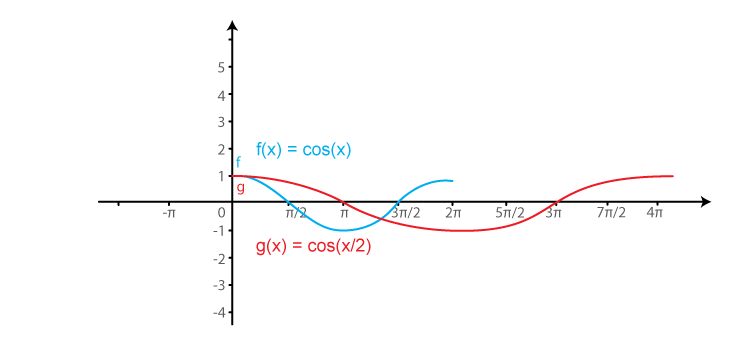(iv) y = cos2 x and y = cos x

We know that the functions y = cos2 x and y = cos x are periodic functions with period 2π.

The values of these functions are tabulated below:

 x 0 π/2 π 3π/2 2π y = cos2 x 1 0 1 0 1 y = cos x 1 0 -1 0 1

The required curve is: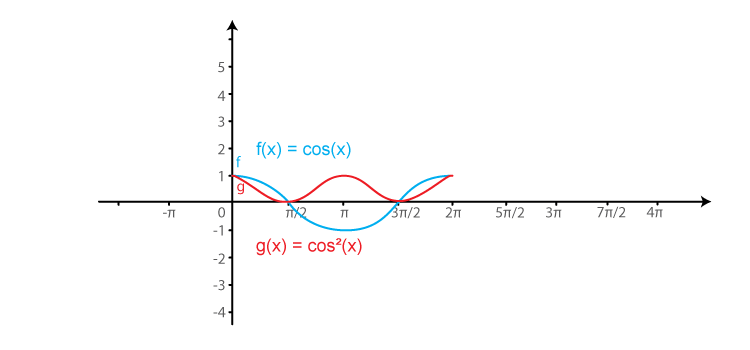EXERCISE 6.3 PAGE NO: 6.13

Sketch the graphs of the following functions:

1. f (x) = 2 cosec πx

Solution:

We know that f (x) = cosec x is a periodic function with period 2π.

So, f (x) = 2 cosec (πx) is a periodic function with period 2. So, we will draw the graph of f (x) = 2 cosec (πx) in the interval [0, 2]. The values of f (x) = 2 cosec (πx) at various points in [0, 2] are listed in the following table:

 x 0 (A) 1/2 (B) 1 (C) -1 (D) 3/2 (E) -2 (F) 2 (G) 5/2 (H) f (x) = 2 cosec (πx) ∞ 2 ∞ -∞ -2 -∞ ∞ 2

The required curve is: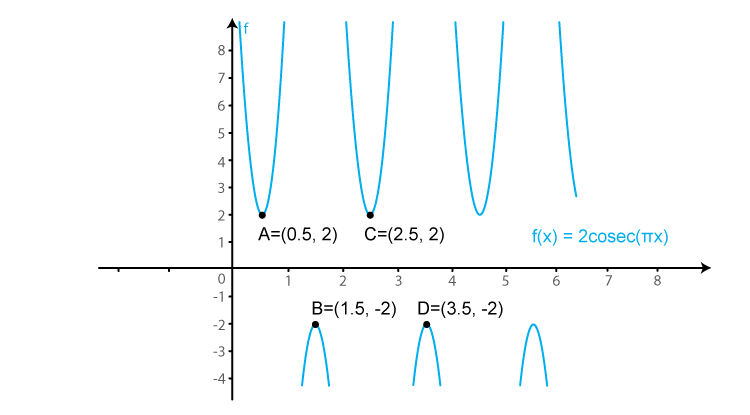2. f (x) = 3 sec x

Solution:

We know that f (x) = sec x is a periodic function with period π.

So, f (x) = 3 sec (x) is a periodic function with period π. So, we will draw the graph of f (x) = 3 sec (x) in the interval [0, π]. The values of f (x) = 3 sec (x) at various points in [0, π] are listed in the following table:

 x 0 (A) π/2 (B) -π/2 (C) π (D) -3π/2 (E) 3π/2 (F) 2π (G) 5π/2 (H) f (x) = sec x 3 ∞ -∞ -3 -∞ ∞ 3 ∞

The required curve is: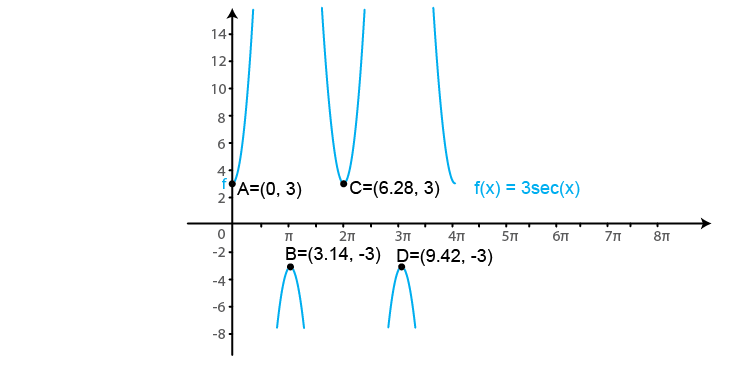3. f (x) = cot 2x

Solution:

We know that f (x) = cot x is a periodic function with period π.

So, f (x) = cot (2x) is a periodic function with period π. So, we will draw the graph of f (x) = cot (2x) in the interval [0, π]. The values of f (x) = cot (2x) at various points in [0, π] are listed in the following table:

 x 0 (A) π/4 (B) -π/2 (C) π/2 (D) 3π/4 (E) -π (F) f (x) = cot x →∞ 0 -∞ →∞ 0 -∞

The required curve is: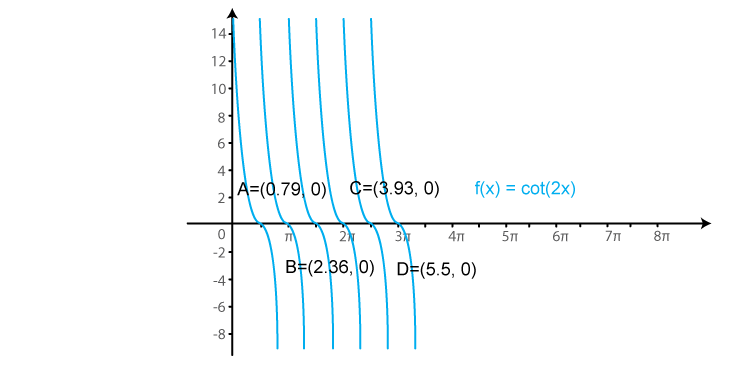4. f (x) = 2 sec πx

Solution:

We know that f (x) = sec x is a periodic function with period π.

So, f (x) = 2 sec (πx) is a periodic function with period 1. So, we will draw the graph of f (x) = 2 sec (πx) in the interval [0, 1]. The values of f (x) = 2 sec (πx) at various points in [0, 1] are listed in the following table:

 x 0 1/2 -1/2 1 -3/2 3/2 2 f (x) = 2 sec (πx) 2 ∞ →-∞ -2 -∞ ∞ 2

The required curve is: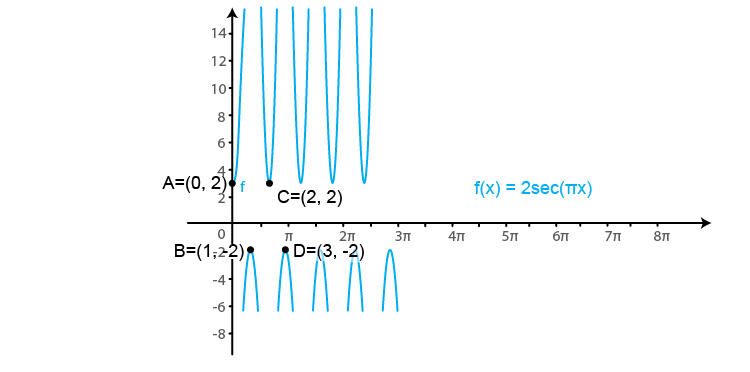5. f (x) = tan2 x

Solution:

We know that f (x) = tan x is a periodic function with period π.

So, f (x) = tan2 (x) is a periodic function with period π. So, we will draw the graph of f (x) = tan2 (x) in the interval [0, π]. The values of f (x) = tan2 (x) at various points in [0, π] are listed in the following table:

 x 0 (A) π/2 (B) π/2 (C) π (D) 3π/2 (E) 3π/2 (F) 2 π f (x) = tan2 (x) 0 ∞ →∞ 0 ∞ →∞ 0

The required curve is: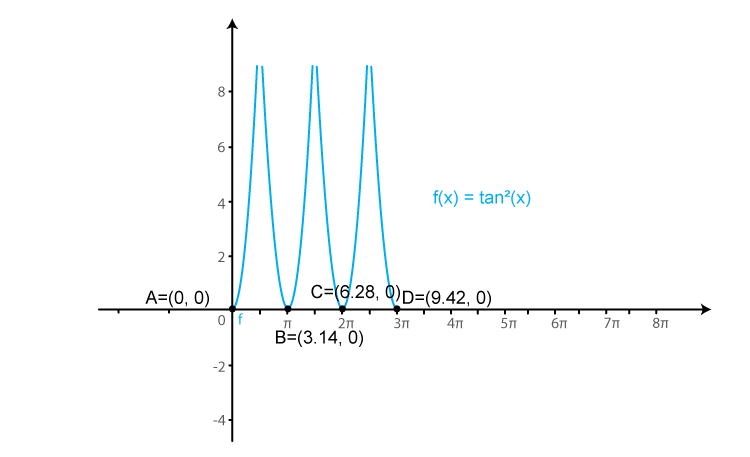## Frequently Asked Questions on RD Sharma Solutions for Class 11 Maths Chapter 6

### List out the frequently asked topics of Chapter 6 of RD Sharma Solutions in the board exam of Class 11 Maths?

The frequently asked topics of Chapter 6 of RD Sharma Solutions in the board exam of Class 11 Maths are
Graph of the sine function.
Graph of the cosine function.
Graph of the tangent function.
Graph of cosecant function.
Graph of cotangent function.
Graph of secant function.

### Why should we download RD Sharma Solutions for Class 11 Maths Chapter 6 from BYJU’S?

BYJU’S provides the most accurate answers for the questions present in the RD Sharma Solutions for Class 11 Maths Chapter 6. These solutions can be viewed online as well as downloaded in the PDF format. The solutions of this chapter are explained by experts very clearly with neat diagrams wherever necessary. The solutions for exercise wise problems are prepared after vast research is conducted on each topic. The step-wise solutions prepared by subject experts help students to solve problems comfortably.

### Is RD Sharma Solutions for Class 11 Maths Chapter 6 important from an exam point of view?

Yes, all the chapters present in RD Sharma Solutions for Class 11 Maths are important for board exams as well as for higher grades. Students should practice all the questions present in RD Sharma Solutions for Class 11 Maths Chapter 6 to procure high marks. By practising the RD Sharma Solutions for Class 11, students will be able to grasp the concepts correctly. Conceptual knowledge is crucial as some of the topics are continued in higher classes.